https://doi.org/10.5194/tc-17-1645-2023
https://doi.org/10.5194/tc-17-1645-2023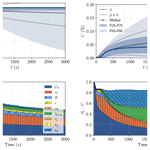# Uncertainty analysis of single- and multiple-size-class frazil ice models

Fabien Souillé, Cédric Goeury, and Rem-Sophia Mouradi
Abstract

The formation of frazil ice in supercooled waters has been extensively studied, both experimentally and numerically, in recent years. Numerical models, with varying degrees of complexity, have been proposed; these are often based on many parameters, the values of which are uncertain and difficult to estimate. In this paper, an uncertainty analysis of two mathematical models that simulate supercooling and frazil ice formation is carried out within a probabilistic framework. The two main goals are (i) to provide quantitative insight into the relative importance of contributing uncertain parameters, to help identify parameters for optimal calibration, and (ii) to compare the output scatter of frazil ice models with single and multiple crystal size classes. The derivation of single- and multi-class models is presented in light of recent work, their numerical resolution is discussed, and a list of the main uncertain parameters is proposed. An uncertainty analysis is then carried out in three steps. Parameter uncertainty is first quantified, based on recent field, laboratory and numerical studies. Uncertainties are then propagated through the models using Monte Carlo simulations. Finally, the relative influence of uncertain parameters on the output time series – i.e., the total frazil volume fraction and water temperature – is assessed by means of Sobol indices. The influence of input parameters on the long-term asymptote as well as short-term transient evolution of the systems is discussed, depending on whether gravitational removal is included or not in the models.

Share
Dates
1 Introduction

Formation of frazil ice in water bodies has been widely investigated because of its impact on submerged structures and because it often precedes formation of ice cover in rivers and oceans . Frazil ice also plays an important role in geophysical flows such as plumes of ice shelf water under floating ice sheets . For these reasons, the study of frazil formation processes is an active area of research, with a large variety of applications in river and coastal engineering.

The main drivers for the formation of frazil are the water cooling rate resulting from heat exchanges with the atmosphere, the initial seeding of frazil nuclei and the turbulent mixing. Then, the thermal growth process deriving from the heat exchange between water and primitive crystals allows a fine description of the balance between growth frazil ice and water supercooling. Previous mathematical models describing the evolution of frazil ice and water temperature were based on ideas pioneered by Daly (1984, 1994). developed a model based on a turbulent channel-flow boundary layer theory, in which a mean particle of constant geometric properties and constant Nusselt number is considered. Mean particle-size models have been incorporated in numerical hydraulic tools such as CRISSP2D . More complex models are based on the ice-number continuity equation introduced by Daly (1984), taking into account the complexity of crystal distribution. In contrast with single-size-class models, the crystal number continuity equations allow introduction of secondary nucleation and flocculation processes. When combined with thermal growth, these complement the modeling of frazil crystal size evolution. proposed a numerical model for solving the equations introduced by Daly (1984) by considering discrete radius intervals. Conceptual models for secondary nucleation and flocculation were introduced and calibrated to fit the experimental data of and . derived a variable particle-size model in two dimensions and later improved it with secondary nucleation and flocculation . Variable particle-size models have also been integrated in TELEMAC-MASCARET . Numerous simplified implementations have been made that consider a well-mixed water body. For example, worked with the same model as and further discussed calibration of influential parameters in initial seeding, secondary nucleation, and flocculation by comparing them with the experimental data of . Implementation of multiple-size-class frazil dynamics in the context of sea ice and ice shelf water plumes has also been proposed by , and . In the same context, have recently shed new light on multiple-size-class models and discussed crystal growth rate and the occurrence of frazil explosion. They also identified and characterized the steady-state crystal size distribution. Henceforth, single- and multiple-size-class models will be referred to as SSC (single size class) and MSC (multiple size class) models respectively.

Common to all numerical model of frazil dynamics is their use of a large number of parameters, making calibration difficult. The modeling studies mentioned above show that frazil ice models can be fitted to reproduce the evolution of temperature and frazil volume fraction. However, given the uncertainty of fitting parameters, it is questionable whether these models are predictive. As point out, the consistency between experiments and models does not necessarily mean parameterization is correct. This is particularly true when different processes compete and have similar impact on the crystal size distribution. Besides, introducing new processes in the models increases the number of parameters that need calibration. This comes at the cost of introducing new uncertain parameters and raises the question of models trustworthiness.

This trade-off between uncertainties and model complexity is often discussed in geophysical and environmental modeling . On this matter advises use of statistics to aid mathematical modeling via “a systemic appraisal of model uncertainties and parametric sensitivities”. There is, moreover, a growing appreciation of how sensitivity analysis enhances the understanding of the intricate physical processes involved in ice formation . The sensitivity of multiple-size-class frazil models to initial seeding, secondary nucleation and flocculation parameters has been investigated by and , by simple modifications of specific parameters. Although probabilistic methods are sometimes used in processing observational data , to the author's knowledge, probabilistic sensitivity analysis of frazil ice models has never been performed. In addition, comparison of SSC and MSC models in terms of uncertainty of output has never been tackled, despite the prohibitive computational cost of the MSC model, making the SSC approach still relevant to many applications.

In this paper, we intend to bridge that gap by proposing an uncertainty and sensitivity analysis of single and multiple-class frazil ice models, using a of full description of parameters uncertainty (by probability density functions) and variance decomposition of model outputs. First, we introduce the models and main hypotheses and discuss their implementation in Sect. 2. The uncertainty assessment methodology is presented in Sect. 3. We then rely on numerous field and experimental measurements to quantify the uncertainties of input parameters of the two frazil numerical models in Sect. 4. Using Monte Carlo experiments, we next study the propagation of these uncertainties on model outputs in Sect. 5: in other words, the evolution of the frazil ice volume fraction and the water temperature. The evolution of output scatter is discussed and compared to the asymptotes of the dynamic systems. Finally, sensitivity analysis based on Sobol indices (Sobol2001) and aggregated Sobol indices enables us to propose a selection of the most influential parameters in both models. We conclude with this study's perspectives in Sect. 6.

2 Frazil ice dynamics

This section introduces the continuum equations used to describe evolution of water temperature and frazil volume fraction, as well as their discrete counterparts and their numerical resolution under the assumption of well-mixed water bodies. The parameters of the models that can be considered uncertain are also introduced.

## 2.1 Mathematical description

Frazil crystals are assumed to be discs of radius r and thickness λ. The ratio between diameter and thickness, denoted $R=\mathrm{2}r/\mathit{\lambda }$, is supposed to be constant as crystals grow. Let us define n as the crystal number density, which corresponds to the number of crystals per unit volume per unit length in radial space. The total number of particles per unit volume is then defined as $N=\underset{\mathrm{0}}{\overset{\mathrm{\infty }}{\int }}n\left(r\right)\mathrm{d}r$. We also introduce the frazil volume fraction density as c=nV, in which V=πr2λ is the crystal volume. The volume proportion of the water–ice mixture occupied by frazil ice, i.e., the total volume fraction of frazil, is then defined as $C=\underset{\mathrm{0}}{\overset{\mathrm{\infty }}{\int }}c\left(r\right)\mathrm{d}r$. An incompressible water–ice mixture of velocity u and depth h is considered. Following Daly (1984) the number density balance equation can be defined as

$\begin{array}{}\text{(1)}& \begin{array}{rl}\frac{\partial n}{\partial t}& +\mathbf{u}\cdot \mathrm{\nabla }n-\mathrm{\nabla }\cdot \left({\mathit{\nu }}_{c}\mathrm{\nabla }n\right)=-\underset{\left(\mathrm{1}\right)}{\underbrace{\frac{\partial }{\partial r}\left(Gn\right)}}\\ & +\underset{\left(\mathrm{2}\right)}{\underbrace{\left({\stackrel{\mathrm{˙}}{N}}_{\mathrm{T}}+{\stackrel{\mathrm{˙}}{N}}_{\mathrm{I}}\right)\mathit{\delta }\left(r-{r}_{c}\right)}}-\underset{\left(\mathrm{3}\right)}{\underbrace{\frac{\mathrm{1}}{V}\frac{\partial }{\partial r}\left(FVn\right)}}-\underset{\left(\mathrm{4}\right)}{\underbrace{{w}_{r}\frac{\partial n}{\partial z}}},\end{array}\end{array}$

where νc is the frazil particle diffusivity, wr is the buoyancy velocity and the remaining right-hand-side terms are source terms described hereafter.

• The source term (1) represents the crystal size evolution due to thermal growth, resulting from the heat exchange between the supercooled water and ice particles. The crystals can be considered to grow mainly on their peripheral area, defined by a=2πrλ (Daly1994). Frazil evolution is then driven by the radial growth rate G, equal to

$\begin{array}{}\text{(2)}& {\mathit{\rho }}_{\mathrm{i}}{L}_{\mathrm{i}}G=\frac{Nu{k}_{\mathrm{w}}}{{\mathit{\delta }}_{\mathrm{T}}}\mathrm{\Delta }T,\end{array}$

in which ρi is the frazil ice density, Li is the latent heat of ice fusion, and the right-hand side is the heat exchange between the crystal of temperature Ti and the surrounding water of temperature T. The latter is modeled as a function of the temperature delta $\mathrm{\Delta }T={T}_{\mathrm{i}}-T$; the thermal conductivity of water kw; the Nusselt number Nu, which represents the ratio between turbulent heat transfer and conduction heat transfer; and a thermal boundary layer length scale, denoted δT (which is either chosen as the radius or thickness in the literature). The crystal temperature Ti is assumed to be equal to the freezing temperature denoted Tf. The Nusselt number can be described as a function of ratio ${m}^{*}=r/\mathit{\eta }$, where η is the Kolmogorov length scale, which can be defined as a function of the turbulent dissipation rate ε as $\mathit{\eta }=\left({\mathit{\nu }}^{\mathrm{3}}/\mathit{\epsilon }{\right)}^{\mathrm{1}/\mathrm{4}}$, in which ν is the molecular viscosity of water (Daly1984). The Nusselt number formulation described by is used. For small particles, i.e., ${m}^{*}\le \mathrm{1}$, heat transfer is governed by diffusion and convection, and the Nusselt number can therefore be written as

where Pr is the Prandtl number, defined as the ratio between molecular and thermal diffusivity. For larger particles (${m}^{*}>\mathrm{1}$), heat transfer is governed by turbulent mixing of the boundary layer around the crystal, and the Nusselt number is defined by

where αT is the turbulent intensity.

• Heterogeneous nucleation (growth from foreign nuclei) and secondary nucleation (birth of new nuclei due to breakage of parent crystals) are invoked to explain the continuous feed of frazil nuclei during the rapid initial frazil growth (Daly1984, 1994). Heterogeneous nucleation is mainly caused by impurities that come either from the water body itself (biological elements, suspended sediments, etc.) or by penetration of new nuclei from the atmosphere (meteorological conditions or artificial seeding during experiments). The source term (2) of Eq. (1) models the introduction of new nuclei, as well as the secondary nucleation, where ${\stackrel{\mathrm{˙}}{N}}_{I}$ is a seeding rate, and ${\stackrel{\mathrm{˙}}{N}}_{T}$ the secondary nucleation rate function of collisions between crystals. The function δ(rrc) is the Dirac delta function, and rc is the critical radius (radius of new nuclei). Note that seeding and secondary nucleation are assumed to introduce particles of the same size. Collisions are supposed to cause small nuclei to break off from parent crystals with a frequency of collision proportional to the crystal velocity relative to the fluid Ur and the total number of particles N that are contained in the volume swept by the crystal . The secondary nucleation rate ${\stackrel{\mathrm{˙}}{N}}_{T}$ is then defined as

$\begin{array}{}\text{(5)}& {\stackrel{\mathrm{˙}}{N}}_{T}=\stackrel{\mathrm{̃}}{n}\underset{\mathrm{0}}{\overset{\mathrm{\infty }}{\int }}\mathit{\pi }{r}^{\mathrm{2}}{U}_{\mathrm{r}}\left(r\right)n\left(r\right)\mathrm{d}r,\end{array}$

where ${U}_{\mathrm{r}}=\sqrt{\frac{\mathrm{4}\mathit{\epsilon }{r}^{\mathrm{2}}}{\mathrm{15}\mathit{\nu }}+{w}_{r}^{\mathrm{2}}}$ is the geometric mean between turbulent velocity and buoyant rise velocity. The number $\stackrel{\mathrm{̃}}{n}=\mathrm{max}\left(N,{n}_{\mathrm{max}}\right)$ is the average number of particles per unit volume that take part in the collisions, and nmax is a fitting parameter controlling the efficiency of the collision breeding. This parametrization was also followed by , , and .

• The source term (3) of Eq. (1) is a flocculation source term supposed to represent the net effect of both flocculation and breakup, as introduced by , who chose F=F0r2, where F0 is a constant. Their choice was motivated by the intuition that larger crystals are more prone to flocculate. However, as explained by , this yields a linear flocculation in the number of crystals. However one could argue that flocculation should be quadratic in the number of crystals like secondary nucleation since it is physically based on the same collision mechanisms. Moreover, it also depends on hydrodynamic conditions, of which the effects on flocculation are still poorly characterized in the literature. To be consistent with models in the literature, the formulation proposed by is chosen for this paper.

• The source term (4) of Eq. (1) represents the buoyancy of frazil ice crystals, where wr is the rise velocity. In the present paper, it is set to wr=32.8(2r)1.2, following , who simplified the model proposed by Daly (1984). For consistency with previous modeling studies, we retain this simple formulation, even if many other formulations have been proposed as summarized by and .

Finally, the thermal balance of the water–ice mixture complements Eq. (1). Assuming C≪0, the water fraction temperature equation is obtained:

$\begin{array}{}\text{(6)}& \frac{\partial T}{\partial t}+\mathbf{u}\cdot \mathrm{\nabla }T-\mathrm{\nabla }\cdot \left({\mathit{\nu }}_{\mathrm{t}}\mathrm{\nabla }T\right)=\frac{\mathit{\varphi }}{\mathit{\rho }{c}_{p}}+\frac{{\mathit{\rho }}_{\mathrm{i}}{L}_{i}}{\mathit{\rho }{c}_{p}}\underset{\mathrm{0}}{\overset{\mathrm{\infty }}{\int }}G\left(r\right)a\left(r\right)n\left(r\right)\mathrm{d}r,\end{array}$

where T is the temperature of the water fraction of the water–ice mixture, νt is the thermal diffusivity, ρ is the density of water, cp is the specific heat of water and ϕ is the net heat source resulting from heat exchanges with the atmosphere in watts per cubic meter (W m−3).

In the following sections, a well-mixed water body is considered. Equations (1) and (6) are written in terms of space-averaged quantities. This assumption allows us to neglect the convection and diffusion operators and to focus on solving the average temperature and average frazil volume fraction. Therefore, the partial differential equations of frazil and temperature are simplified to a coupled set of ordinary differential equations (ODEs) including only the source terms.

In this section we introduce a multiple-size-class MSC frazil ice model, which is a discrete version of Eqs. (1) and (6) in radial space. It consists of considering m classes of constant radius chosen between a minimum and a maximum radius . For each class i, the radius and the thickness are supposed to be equal to ri and ${\mathit{\lambda }}_{i}=\mathrm{2}{r}_{i}/R$ ($\mathrm{1}\le i\le m$). The peripheral area as well as the surface and volume of frazil crystals are defined as ${a}_{i}=a\left({r}_{i}\right)=\mathrm{2}\mathit{\pi }{r}_{i}{\mathit{\lambda }}_{i}$, ${s}_{i}=s\left({r}_{i}\right)=\mathrm{2}\mathit{\pi }\left({r}_{i}{\mathit{\lambda }}_{i}+{r}_{i}^{\mathrm{2}}\right)$ and ${V}_{i}=V\left({r}_{i}\right)=\mathit{\pi }{r}_{i}^{\mathrm{2}}{\mathit{\lambda }}_{i}$, respectively. A log-uniform discretization of the radial space is chosen as in previous studies . The number of crystals of class i is denoted ni, and, similarly, the volume fraction of crystals of class i is denoted ci. The total volume fraction of frazil and total number of particles are then defined as $C=\sum _{i=\mathrm{1}}^{m}{c}_{i}$ and $N=\sum _{i=\mathrm{1}}^{m}{n}_{i}$ with ci=niVi, respectively. The discrete version of Eq. (1) written in terms of volume fraction balance reads

$\begin{array}{}\text{(7)}& \begin{array}{rl}\frac{\mathrm{d}{c}_{i}}{\mathrm{d}t}& =\underset{\left(\mathrm{1}\right)}{\underbrace{{V}_{i}\left({\mathrm{\Gamma }}_{i-\mathrm{1}}{c}_{i-\mathrm{1}}+\left({\mathrm{\Lambda }}_{i}-{\mathrm{\Gamma }}_{i}\right){c}_{i}-{\mathrm{\Lambda }}_{i+\mathrm{1}}{c}_{i+\mathrm{1}}\right)}}+\underset{\left(\mathrm{2}\right)}{\underbrace{{\mathit{\tau }}_{i}}}\\ & +\underset{\left(\mathrm{3}\right)}{\underbrace{{\mathit{\beta }}_{i-\mathrm{1}}{c}_{i-\mathrm{1}}-{\mathit{\beta }}_{i}{c}_{i}}}-\underset{m}{\underbrace{{\mathit{\gamma }}_{i}{c}_{i}}}\left(\mathrm{4}\right)\phantom{\rule{0.25em}{0ex}}\left(\mathrm{1}\le i\le m\right),\end{array}\end{array}$

with the boundary conditions ${V}_{\mathrm{0}}={V}_{m+\mathrm{1}}={\mathrm{\Gamma }}_{\mathrm{0}}={\mathrm{\Gamma }}_{m}={\mathrm{\Lambda }}_{m+\mathrm{1}}={\mathit{\beta }}_{\mathrm{0}}={\mathit{\beta }}_{m}=\mathrm{0}$. The discrete versions of the source terms introduced in Eq. (1) are defined following previous work .

• The thermal growth source term (1) is composed of thermal growth and melt functions Γi and Λi, which are defined as

$\begin{array}{}\text{(8)}& & {\mathrm{\Gamma }}_{i}=H\frac{{G}_{i}{a}_{i}}{{V}_{i}\mathrm{\Delta }{V}_{i}},\text{(9)}& & {\mathrm{\Lambda }}_{i}=\left(\mathrm{1}-H\right)\frac{{G}_{i}{s}_{i}}{{V}_{i}\mathrm{\Delta }{V}_{i-\mathrm{1}}},\end{array}$

where Gi=G(ri), $\mathrm{\Delta }{V}_{i}={V}_{i+\mathrm{1}}-{V}_{i}$ and $H={H}_{e}\left({T}_{\mathrm{f}}-T\right)$, with He the Heaviside function allowing a switch between melting or freezing. We assume that frazil crystals grow from their peripheral area ai but melt from their surface si .

• The crystal birth source term (2), composed of the secondary nucleation and seeding, reads

$\begin{array}{}\text{(10)}& & {\mathit{\tau }}_{i\ne \mathrm{1}}=-\mathit{\zeta }{\mathit{\alpha }}_{i}{c}_{i},\text{(11)}& & {\mathit{\tau }}_{i=\mathrm{1}}={\mathit{\tau }}_{\mathrm{s}}{V}_{\mathrm{1}}/h+\sum _{j=\mathrm{2}}^{m}{\mathit{\alpha }}_{j}{c}_{j},\end{array}$

where $\mathit{\zeta }={V}_{\mathrm{1}}/{V}_{i}$ is a coefficient to conserve crystal volume; ${\mathit{\alpha }}_{i}=\mathit{\pi }\stackrel{\mathrm{̃}}{n}{U}_{\mathrm{r}}\left({r}_{i}\right){r}_{i}^{\mathrm{2}}$; $\stackrel{\mathrm{̃}}{n}=\mathrm{max}\left(N,{n}_{\mathrm{max}}\right)$, with nmax a fitting parameter for collision breeding; and τs is a constant seeding rate in m−2 s−1.

• The flocculation source term (3) is defined as ${\mathit{\beta }}_{i}={a}_{\mathrm{f}}{r}_{i}/{r}_{\mathrm{1}}$, where af is a flocculation coefficient in s−1.

• The buoyancy of crystals is simplified into a gravitational removal sink term (4) defined as ${\mathit{\gamma }}_{i}=-{w}_{r}\left({r}_{i}\right){a}_{d}/h$, in which h is the water depth. We introduce a coefficient ad to account for the uncertainty of the rise velocity and gravitational removal process.

Finally, writing the thermal growth source term as ${S}_{i}={V}_{i}\left({\mathrm{\Gamma }}_{i-\mathrm{1}}{c}_{i-\mathrm{1}}+\left({\mathrm{\Lambda }}_{i}-{\mathrm{\Gamma }}_{i}\right){c}_{i}-{\mathrm{\Lambda }}_{i+\mathrm{1}}{c}_{i+\mathrm{1}}\right)$, one can write the discrete version of Eq. (6) as

$\begin{array}{}\text{(12)}& \frac{\mathrm{d}T}{\mathrm{d}t}=\frac{\mathit{\varphi }}{\mathit{\rho }{c}_{p}}+\frac{{\mathit{\rho }}_{\mathrm{i}}{L}_{i}}{\mathit{\rho }{c}_{p}}\sum _{i=\mathrm{1}}^{m}{S}_{i}.\end{array}$

A general discrete frazil ice model is implemented in the present work (Eqs. 7 and 12) so that one can easily retrieve the formulations developed in previous papers. For example, supposing H=1, ad=1, and τs=0 and writing ci=niVi, Eq. (7) is equivalent to Eq. (4) in and Eq. (1) in with ζ=1. By also neglecting flocculation (af=0), it is equivalent to Eq. (10) in .

## 2.3 Single-size-class simplification

A simplified approach is to take a single-size-class SSC composed only of particles of radius $\stackrel{\mathrm{‾}}{r}$ representative of the whole crystal distribution. A simplified set of equations for the frazil volume fraction and water temperature can then be written as

$\begin{array}{}\text{(13)}& & \frac{\mathrm{d}C}{\mathrm{d}t}=GaN+\frac{\mathrm{1}}{h}\left(V{\mathit{\tau }}_{\mathrm{s}}-{w}_{r}{a}_{d}C\right),\text{(14)}& & \frac{\mathrm{d}T}{\mathrm{d}t}=\frac{\mathit{\varphi }}{\mathit{\rho }{c}_{p}}+\frac{{\mathit{\rho }}_{\mathrm{i}}{L}_{i}}{\mathit{\rho }{c}_{p}}GaN,\end{array}$

where $a=a\left(\stackrel{\mathrm{‾}}{r}\right)$, $V=V\left(\stackrel{\mathrm{‾}}{r}\right)$, ${w}_{r}={w}_{r}\left(\stackrel{\mathrm{‾}}{r}\right)$ and $G=G\left(\stackrel{\mathrm{‾}}{r}\right)$, with G(r) being defined by Eq. (2) and the Nusselt number by Eqs. (3) and (4). In the previous equations, τs is the seeding rate, ad is the buoyancy coefficient, h is the water depth and wr is the buoyancy velocity. The SSC system can either be expressed in terms of total number of frazil crystals N or total frazil volume fraction C using the expression $N=C/V$.

## 2.4 Numerical methods to solve governing equations

A semi-implicit theta scheme is implemented for the MSC model, with a constant time step Δt, and a fully implicit method is used for the SSC model. Details on the numerical resolution are provided in Appendix A. Note that the semi-implicit time scheme is subject to a stability condition function of the smallest radius. In the present study, we found that, for the range of radius tested in the sensitivity analysis, decreasing the time step below Δt=0.25 s did not impact the results, so a value of 0.25 s was retained for all simulations.

An important aspect of the numerical frazil ice models presented in this paper is the need to provide a non-zero initial condition for the frazil volume fraction (in absence of seeding: i.e., τs=0). In the case of MSC models, assumed a uniform initial number of particles n0 in each radius class, i.e., ni(t0)=n0 ($\mathrm{1}\le i\le m$). We followed the same principle to initialize the system but fixed the number of initial particles at zero for classes with a radius exceeding a threshold r0: i.e., ${n}_{{r}_{i}\le {r}_{\mathrm{0}}}\left({t}_{\mathrm{0}}\right)={n}_{\mathrm{0}}$ like proposed in other works . To be able to compare SSC and MSC models, we worked in terms of initial volume fraction of frazil C(t0)=C0. The system was then initialized with ${n}_{\mathrm{0}}={C}_{\mathrm{0}}/\sum _{{r}_{i}\le {r}_{\mathrm{0}}}{V}_{i}$ and ci(t0)=ni(t0)Vi ($\mathrm{1}\le i\le m$). As the initial setup has a significant influence on the results , C0 and r0 are considered in the following sections as uncertain parameters.

To test convergence in terms of the number of radius classes, we make it vary from m=10 to m=200. The results, presented in Fig. 1, are consistent with observations by that the system requires m≳100 to converge. Note that and took m=20 and m=40, respectively. It should also be noted that convergence in the number of classes depends on the initialization method of the system, as highlighted by . To perform all the numerical simulations required for a complete sensitivity analysis, m=100 was chosen as a trade-off between numerical convergence and computational cost.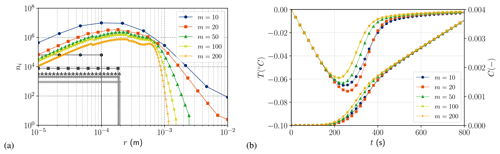Figure 1MSC model convergence in the number of classes with ${C}_{\mathrm{0}}=\mathrm{4.5}×{\mathrm{10}}^{-\mathrm{8}}$, r0=0.2 mm, ${r}_{\mathrm{min}}={\mathrm{10}}^{-\mathrm{5}}$ m, ${r}_{\mathrm{max}}={\mathrm{10}}^{-\mathrm{2}}$ m, dt=0.1 s, $\mathit{\varphi }=-\mathrm{1400}$ W m−3, $\mathit{\epsilon }=\mathrm{1.2}×{\mathrm{10}}^{-\mathrm{3}}$ m2 s−3, αT=0.0876, ${n}_{\mathrm{max}}=\mathrm{8}×{\mathrm{10}}^{\mathrm{6}}$ m−1, ${a}_{\mathrm{f}}={\mathrm{10}}^{-\mathrm{4}}$ s−1, τs=0 m−2 s−1 and ad=0. Number of crystals per class (a) at initial time (black) and at time t=300 s (color). Water temperature and frazil volume fraction vs. time (b).

## 2.5 Study cases and model parameters

In the present study, we focus on the evolution of water temperature and total frazil volume fraction in a supercooled, well-mixed water body of depth h=1 m. To focus on the frazil modeling rather than heat budget, uncertainties deriving from exchanges with the atmosphere are neglected and the cooling rate ϕ is considered deterministic and constant over time in all experiments. Nonetheless, different values of ϕ were tested, ranging from −50 to −1000 W m−3, to test the variability of the results in different cooling rate situations. As described in previous sections, frazil ice models are driven by many parameters, some of which are subject to a significant degree of uncertainty. The list of parameters considered in the present study for conducting the uncertainty analysis is shown in Table 1. Some physical properties are considered constant and are taken at T=0C such that $\mathit{\nu }=\mathrm{1.792}×{\mathrm{10}}^{-\mathrm{6}}$ m2 s−1, ρ=999.82 kg m−3, ρi=916.8 kg m−3, ${L}_{i}=\mathrm{3.35}×{\mathrm{10}}^{\mathrm{5}}$ J kg−1, ${c}_{p}=\mathrm{4.1855}×{\mathrm{10}}^{\mathrm{3}}$ J kg−1 K−1 and kw=0.561 W m−1 K−1.

Taking the SSC model as a reference, one can simply visualize the main feature of frazil dynamic systems. When time is close to zero, the initial temperature decrease rate is defined by $\underset{t\to \mathrm{0}}{lim}\stackrel{\mathrm{˙}}{T}=\mathit{\varphi }/\mathit{\rho }{c}_{p}$. In the absence of seeding and gravitational removal (i.e., τs=0 and ad=0), the temperature decreases to the maximum supercooling point, characterized by a maximal temperature depression, denoted $\mathit{\theta }=\mathrm{max}\left({T}_{\mathrm{f}}-T\right)$, and a critical time, denoted tc. After the maximum supercooling, the frazil production rate releases more heat compared to that released in the atmosphere, leading to an increase in water temperature which tends toward freezing point. Finally, when time tends to infinity, there is a balance between the frazil growth source term and the cooling rate. This leads to a linear increase in frazil volume fraction at a constant rate, i.e., $\underset{t\to +\mathrm{\infty }}{lim}\stackrel{\mathrm{˙}}{C}=-\mathit{\varphi }/{\mathit{\rho }}_{\mathrm{i}}{L}_{i}$. This typical evolution of water temperature and frazil volume fraction is described in Fig. 2, in which the frazil volume fraction asymptote, denoted C, is defined as

$\begin{array}{}\text{(15)}& {C}^{\mathrm{\infty }}={C}_{\mathrm{0}}-\mathit{\varphi }t/{\mathit{\rho }}_{\mathrm{i}}{L}_{i}.\end{array}$

By introducing seeding and gravitational removal, i.e., τs≠0 and ad≠0, the frazil ice long-term asymptote is modified, and we have $\underset{t\to +\mathrm{\infty }}{lim}\stackrel{\mathrm{˙}}{C}=-\mathit{\varphi }/{\mathit{\rho }}_{\mathrm{i}}{L}_{i}+\left(V{\mathit{\tau }}_{\mathrm{s}}-{w}_{r}{a}_{d}C\right)/h$. Considering ϕ and τs as constant over time, this yields a convergence of the frazil volume fraction towards a finite limit, defined by the ratio between the buoyancy removal and the production rate due to thermal growth and seeding, which reads

$\begin{array}{}\text{(16)}& {C}^{\mathrm{\infty }}=\frac{h}{{a}_{d}{w}_{r}}\left(-\frac{\mathit{\varphi }}{{\mathit{\rho }}_{\mathrm{i}}{L}_{i}}+\frac{V{\mathit{\tau }}_{\mathrm{s}}}{h}\right).\end{array}$

It should be observed that the steady states are not affected by the crystal growth rate. Similar observations were discussed by for the MSC model.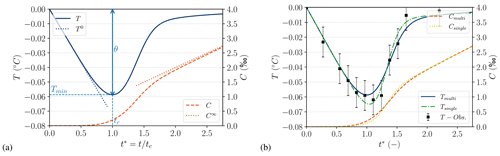Figure 2Typical evolution of water temperature and frazil volume fraction vs. time (a) and reproduction of case 1 experiments (b) with dt=0.1 s, $\mathit{\varphi }=-\mathrm{1400}$ W m−3, $\mathit{\epsilon }=\mathrm{1.2}×{\mathrm{10}}^{-\mathrm{3}}$ m2 s−3, αT=0.0876, ${n}_{\mathrm{max}}=\mathrm{8}×{\mathrm{10}}^{\mathrm{6}}$ m−1, ${a}_{\mathrm{f}}={\mathrm{10}}^{-\mathrm{4}}$ s−1, τs=0 m−2 s−1, ad=0, $\stackrel{\mathrm{‾}}{r}=\mathrm{3.8}×{\mathrm{10}}^{-\mathrm{4}}$ m, R=10, ${C}_{\mathrm{0}}=\mathrm{5.1}×{\mathrm{10}}^{-\mathrm{6}}$ (SSC) and ${C}_{\mathrm{0}}=\mathrm{4.5}×{\mathrm{10}}^{-\mathrm{8}}$ (MSC), r0=0.2 m, ${r}_{\mathrm{min}}={\mathrm{10}}^{-\mathrm{5}}$ m, ${r}_{\mathrm{max}}={\mathrm{10}}^{-\mathrm{3}}$ m, m=100, and δT=λ.

In the following the recovery time is defined as the time when temperature has recovered 90 % of its supercooling depression. We refer to the recovery phase to describe the long-term evolution of the system, i.e., all times past recovery time. Similarly, we will refer to the transient phase as times between initial time and recovery time.

In the present study, we analyzed the uncertainty of input parameters in the main transient and steady-state features of the frazil ice models with and without gravitational removal. We started the simulation with a water temperature equal to the freezing temperature (which is supposed to be equal to zero in the absence of salinity: i.e., Tf=0C). We checked that all the simulations ran beyond the critical time. For the range of parameters considered there, it was found that choosing a final time of tf≃1 h was enough to capture the transient features of the ODE systems.

Note that both the SSC and MSC models are able to reproduce the experiments of , and as shown by and . In Fig. 2 we give an example of the results of both SSC and MSC models compared to experimental results (case 1).

3 Probabilistic framework for uncertainty analysis

The objective of this section is to describe the mathematical tools necessary to study the uncertainties of SSC and MSC frazil ice models. An uncertainty study of a numerical model can be performed in three steps (Sudret2007). The first step consists in identifying the uncertain parameters and characterizing the probabilities of occurrence for their values through probability density functions (PDFs), which is referred to as quantification of uncertainty sources. The second step concerns the propagation of uncertainties through the interest models, generally using sampling techniques (e.g., Monte Carlo) to obtain possible values of the target outputs (here frazil ice concentration, water temperature) and compute their statistical estimates (mean, standard deviation, percentiles, etc.). The third step, called sensitivity analysis, focuses on ranking the uncertain parameters in terms of influence on the target output. In the following, formulations for the statistical estimates and sensitivity analysis indices are given, with a summary of elements essential to understand this paper. Theoretical details can be found in and . All uncertainty analysis computations were performed with the OpenTURNS library (version 1.18) developed by a partnership between Airbus Group, EDF R & D, IMACS, ONERA and Phimeca .

## 3.1 Uncertainty quantification

Let $\mathbit{X}=\left({X}^{\mathrm{1}},\phantom{\rule{0.125em}{0ex}}\mathrm{\dots },\phantom{\rule{0.125em}{0ex}}{X}^{{n}_{X}}\right)$ be the vector of uncertain parameters of the frazil ice model, where nX is the number of uncertain parameters. Let g be the interest frazil model, either SSC or MSC models presented in Eqs. (14) and (12) for temperature and Eqs. (13) and (7) for frazil volume fraction. The output of the interest models is the frazil volume fraction and water temperature discrete time series, i.e., T(tk) and C(tk) ($\mathrm{1}\le k\le {n}_{t}$), which we will generally denote $\mathbit{Y}=\left({Y}^{\mathrm{1}},\phantom{\rule{0.125em}{0ex}}\mathrm{\dots },\phantom{\rule{0.125em}{0ex}}{Y}^{{n}_{t}}\right)$ below for the sake of simplicity. The random vectors X and Y are linked through frazil models g such that $\mathbit{Y}=g\left(\mathbit{X},\mathbit{d}\right)$, where d is a deterministic vector, i.e., fixed parameters in contrast with the uncertain set of inputs X.

To undertake the uncertainty studies, both the inputs X and the interest outputs Y are considered to be random vectors. We assume that X has a density pX, so that $\mathbb{P}\left(\mathbit{X}\in E\subseteq {D}_{\mathbit{X}}\right)=\underset{E}{\int }{p}_{\mathbit{X}}\left(\mathbit{x}\right)d\mathbit{x}$, where E is a subset from the space of all possible values DX. Each element Xi and Yk of the input and output vectors is hence characterized by a PDF.

The results of the uncertainty analysis are directly linked to the UQ (uncertainty quantification) study specification and consequently to the description of the uncertain input parameters X. Thus, special attention is paid to propose a relevant quantification of uncertainty sources. This particular point is addressed in Sect. 4, in which frazil literature is explored to provide adequate bounds and PDFs for each parameter identified in Table 1.

## 3.2 Uncertainty propagation of random variables

In the following, we suppose that PDFs of inputs X are known, in contrast to those of Y. The objective is therefore to characterize the latter by estimating statistical indicators as the PDF's mean and standard deviation. To achieve this, a Monte Carlo sampling method can be used.

In the Monte Carlo method, we generate a sample of independent observations of the random vector X using the joint PDF of the input random vector. For each observation of the input X, we evaluate the corresponding output Y. The resulting experimental design of the input random vector X is an array of size N×nX, where each row, denoted ${\mathbit{x}}_{i}=\left\{{x}^{\mathrm{1}},\mathrm{\dots },{x}^{{n}_{X}}\right\}$, represents a possible configuration of the frazil model. The output realizations, i.e., ${\mathbit{y}}_{i}=\mathit{\left\{}{y}_{i}^{\mathrm{1}},\phantom{\rule{0.125em}{0ex}}\mathrm{\dots },\phantom{\rule{0.125em}{0ex}}{y}_{i}^{{n}_{t}}\mathit{\right\}}$, are generated by N deterministic simulations with corresponding inputs. For a sample of size N, the output of the model is a N×nt array: $\mathbit{Y}=\left[{y}_{i}^{k}{\right]}_{i,k}=\left[{g}^{k}\left({\mathbit{x}}_{i},d\right){\right]}_{i,k}\in {\mathbb{R}}^{N×{n}_{t}}$. Each row of the matrix represents one output time series for a given set of input parameters, while each column represents the output realizations at a given time tk.

Statistical estimators of the response can then be computed. The Monte Carlo estimation of the mean, denoted ${\stackrel{\mathrm{^}}{\mathit{\mu }}}_{{Y}^{k}}$, and standard deviation, denoted ${\stackrel{\mathrm{^}}{\mathit{\sigma }}}_{{Y}^{k}}$, at time tk reads

$\begin{array}{}\text{(17)}& {\stackrel{\mathrm{^}}{\mathit{\mu }}}_{{Y}^{k}}=\frac{\mathrm{1}}{N}\sum _{j=\mathrm{1}}^{N}{y}_{j}^{k}\phantom{\rule{0.25em}{0ex}}\mathrm{and}\phantom{\rule{0.25em}{0ex}}{\stackrel{\mathrm{^}}{\mathit{\sigma }}}_{{Y}^{k}}=\sqrt{\frac{\mathrm{1}}{N}\sum _{j=\mathrm{1}}^{N}{\left({y}_{j}^{k}-{\stackrel{\mathrm{^}}{m}}_{{Y}^{k}}\right)}^{\mathrm{2}}}.\end{array}$

These estimations converge to the true values following the law of large numbers (conditioned by the existence of corresponding PDF's first and second moment, i.e., expectation and variance; ). The convergence order of the Monte Carlo method is given by the central limit theorem, leading to a decrease in the confidence intervals' sizes proportional to $\mathrm{1}/\sqrt{N}$. In the results of this paper, confidence intervals of the estimated mean and standard deviation are computed using a bootstrap method.

## 3.3 Sensitivity analysis using Sobol indices

Sensitivity analysis is essential in understanding numerical models . It aims at quantifying the impact of input variable imprecision on the accuracy of the model output variables. Conventional approaches to global sensitivity analysis (GSA) imply the stochastic estimation of statistical moments and indices classically achieved with the Monte Carlo technique. In the present work, the relative influence of uncertain parameters on the output time series is assessed by means of Sobol indices resulting from the ANOVA (analysis of variance) variance decomposition (Sobol2001). Sobol indices are computed via a modified version of the method proposed by , which is described in Appendix B. For each time step tk ($\mathrm{1}\le k\le {n}_{t}$) and $i\in \mathit{\left\{}\mathrm{1},\phantom{\rule{0.125em}{0ex}}\mathrm{\dots },\phantom{\rule{0.125em}{0ex}}{n}_{X}\mathit{\right\}}$, first-order and total Sobol indices, denoted ${S}_{i}^{k}$ and $S{T}_{i}^{k}$ respectively, are computed. By definition, at any time tk, the total Sobol sensitivity index ($S{T}_{i}^{k}$) measures the contribution to the output variance of the uncertain variable Xi, including all variance caused by its interaction, of any order, with any other input variables. Thus, the variance part explained by variable interactions of the input factor Xi with the other uncertain parameters is determined by subtracting the total Sobol sensitivity index ($S{T}_{i}^{k}$) and the first-order Sobol index (${S}_{i}^{k}$) characterizing the influence of the variable Xi alone. Finally, the discrete time first-order and total Sobol indices are aggregated, denoted ASi and ASTi respectively, as proposed by .

4 Uncertainty source quantification

Many laboratory experiments have been carried out to better understand frazil ice dynamics as summed up by . These experiments, as well as field measurements, help us define parameter variability. In this section, we consider the uncertain parameters listed in Table 1 and deduce adequate variability and PDFs for uncertainty propagation and sensitivity analysis using the aforementioned data. The main geometrical properties of crystals and radial space discretization are first discussed, as well as initial concentration. We then review all the parameters involved in frazil source terms in the same chronology as presented in Sect. 2.

## 4.1 Crystals' geometrical properties

Description of the frazil crystals' shape has been the subject of several field and laboratory measurements (Arakawa1954; Daly1984, 1994). The crystals have been described as thin discs that grow mainly from their peripheral area. More recently, photos have brought valuable confirmation of the disc shape of frazil crystals but also highlighted the complexity in larger flocs' geometry . Let us recall that, in both SSC and MSC models, discretization is done in radial space. Therefore, either the ratio R or the thickness λ must be considered constant to fully describe disc-shaped particles. In the present study, frazil crystals are assumed to have the same aspect ratios, which is consistent with previous studies' hypotheses . Note that considered constant thickness instead but highlighted a weak dependency of the thermal growth on the aspect ratio. Let us discuss the uncertainties associated with the choice of the radius discretization as well as the diameter-to-thickness ratio.

Either a mean radius or a radius space discretization must be specified for SSC and MSC models, respectively. In both cases, observed radius distribution and particle size, reported in a many studies, are taken into consideration. For the sake of synthesis, we analyzed the corresponding publications from 1950 to 2019 and report the available observations in Fig. 3. This figure is complementary to Table 7 of , who summarize particle sizes from both laboratory and field measurements. and reported log-normal distributions of particles in laboratory experiments, with a mean radius ranging from 0.12 to 0.25 mm and 0.49 to 1.4 mm, respectively. This was later confirmed by and in the University of Alberta cold room facility, with mean size ranging from 0.66 to 0.94 mm. recently published similar results under different salinities, with mean radius ranging from 0.45 to 0.52 mm. Log-normal distributions were also observed in field studies, with the mean ranging from 0.59 to 0.94 mm in a report by . Gathering together all reported mean radius ranges, an uncertainty interval of $\mathrm{1.2}×{\mathrm{10}}^{-\mathrm{4}}$ to $\mathrm{2.1}×{\mathrm{10}}^{-\mathrm{3}}$ m was chosen for the mean radius in the SSC model. When their full variation range is considered, frazil crystal sizes are known to follow a log-normal distribution as previously argued, but there is no evidence or reason for the mean radius being log-normal as well. The number of field and laboratory observations is still too small to fit an empirical PDF on the mean radius with reasonable confidence. Hence, a log-uniform PDF is chosen with the previously described bounds. Using log-uniform approximate distributions allows us to explore by means of evenly distributed values the parameters that vary over several orders of magnitude.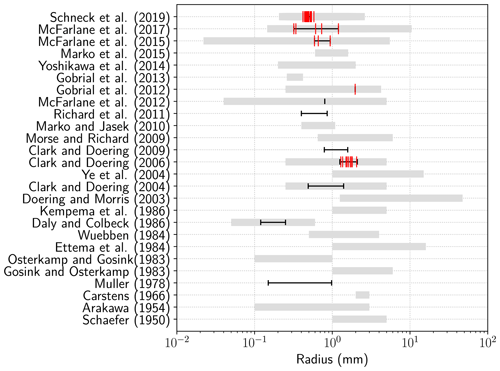Figure 3Particle-size ranges (thick grey line), mean range (thin dark line) and log-normal distributions' mean radius (red vertical ticks) reported in field or laboratory experiments .

For the MSC model, both a minimum and a maximum radius are to be determined for the bounds of the radial discretization. The minimum radius can be determined from survival theory and is about 4 µm (Mercier1984). The maximum size of frazil particles is about 1 to 5 mm according to , but frazil flocs can be significantly larger as shown by and can range up to several centimeters . worked with [$\mathrm{4}×{\mathrm{10}}^{-\mathrm{6}}$, $\mathrm{3}×{\mathrm{10}}^{-\mathrm{3}}$] m while and chose [$\mathrm{7}×{\mathrm{10}}^{-\mathrm{5}}$, $\mathrm{5}×{\mathrm{10}}^{-\mathrm{3}}\right]$ m and [$\mathrm{5}×{\mathrm{10}}^{-\mathrm{5}}$, $\mathrm{5}×{\mathrm{10}}^{-\mathrm{2}}$] m, respectively. In the literature, rmin is taken to be between 10−6 and 10−4, so we chose an intermediate order of magnitude of ${r}_{\mathrm{min}}={\mathrm{10}}^{-\mathrm{5}}$ m. The same choice was made for the maximum radius, leading to a value of ${r}_{\mathrm{max}}={\mathrm{10}}^{-\mathrm{3}}$ m. Both parameters were then considered as uncertain parameters with log-uniform PDFs and were taken to be within [10−6, 10−4] for rmin and within [10−3, 10−1] for rmax.

The aspect ratio ranged from 6 to 13 in the study by , while there were greater values, from 12.2 to 16.33, in . reported an even wider aspect ratio range, from 5 to 100. More recently, reported aspect ratios ranging from 11 to 71 with a mean of 37 and a standard deviation of 11. Considering all these observations, an uncertainty range of 5 to 100 was chosen for the diameter-to-thickness ratio. As not enough data to properly fit a PDF on aspect ratios were provided, a uniform PDF is chosen for the sensibility analysis (maximum entropy principle; ).

Weak dependency of the thickness on the radius was reported by , who suggest assuming a increasing aspect ratio as discs grow instead of a constant aspect ratio. Note also that, as frazil ice forms larger flocs, the disc shape hypothesis commonly accepted in models does not hold anymore. This could lead to erroneous estimations of the thermal growth rate of larger flocs. However, neither the variability of the aspect ratio in the crystals' distribution nor that of the shape is considered in the present study, and we make the assumption that r and R are independent.

## 4.2 Initial concentration

As previously mentioned, either a non-zero initial volume fraction or a non-zero seeding rate is required to trigger thermal growth in the model. In experimental facilities, frazil ice nuclei are sometimes artificially introduced in water to initiate frazil ice growth , but the initial concentration is rarely addressed and depends on the method of initial seeding. As such, a large uncertainty domain is considered in this study for the initial volume fraction of frazil, ranging from 10−8 to 10−4, to account for the lack of data on this parameter. Additionally, a log-uniform PDF is retained since the parameter range variation varies over several orders of magnitude.

For the MSC model, it is necessary to choose how the initial volume fraction is distributed over size classes. One possibility is to initialize the system with log-uniform distributions, similar to the one observed in laboratory experiments. However, observations of nuclei close to rc in size are limited. Consequently, authors have preferred simpler initialization methods, whereby a constant number of crystals is distributed equally over all classes . argued that distributing the initial volume fraction over a range of radii, thus changing the initial number of particles per class, significantly impacts results. They found that filling only the first size class, as in , has less impact on results and should therefore be the preferred initialization method. Given poor evidence of initial predominance of each class in nature, we decided to test both initialization methods, i.e., distributing the initial volume fraction over a range of small radii as presented in Sect. 2.4 and then feeding only the first class as suggested by . For the first method, ice nuclei are supposed to be initially spread between the minimum radius rmin and a threshold radius r0, which we suppose can only be smaller than the mean radius (see Fig. 3). Therefore, the threshold r0 is considered uncertain within the bounds [$\mathrm{1.2}×{\mathrm{10}}^{-\mathrm{4}}$, $\mathrm{2.1}×{\mathrm{10}}^{-\mathrm{3}}$]. For the second method, we set r0=rmin, so that only the first receives the initial concentration.

## 4.3 Thermal growth and turbulent parameters

As shown in Eq. (2), thermal growth (1) is mainly affected by two uncertain parameters, which are the Nusselt number and the thermal boundary layer thickness. In this section, we discuss the uncertainty of both parameters. Since the Nusselt number is being modeled via turbulent parameters, namely the turbulent dissipation rate and the turbulent intensity, their uncertainty is also addressed.

As discussed by , the thermal boundary layer thickness δT is not constant around a crystal, and in recent studies there has been an inconsistent scaling of thermal growth. chose the length scale as δT=λ, while others have chosen δT=r , leading to a serious underestimation of the growth rate, since λ<r for frazil discs. have shown that there is a logarithmic dependency of the thermal growth on the aspect ratio that favors the δT=λ scaling. To account for the variability of the thermal boundary layer thickness, δT should be taken as an uncertain parameter. However, it should be mentioned that, with the variation range being ${\mathit{\delta }}_{\mathrm{T}}\in \left[\mathit{\lambda },r\right]$, the ANOVA methodology (Sect. 3.3) cannot be applied, since the set of uncertain inputs, which contains δT and $\mathit{\lambda }/r$, cannot be considered independent anymore. To overcome this difficulty, the sensitivity analysis is conducted with the most appropriate scaling: i.e., δT=λ, for both the SSC and MSC models. Nevertheless, we propose to investigate the impact of scaling by considering ${\mathit{\delta }}_{\mathrm{T}}\in \left[\mathrm{min}\left(\mathit{\lambda }\right),\mathrm{max}\left(r\right)\right]$ to avoid modeling dependency. A log-uniform PDF is used, and min(λ) and max(r) are estimated from their full variation ranges. A third experiment was also carried out with the δT=r scaling. The three results are then compared for the SSC model.

Two main turbulence parameters are considered: turbulent dissipation rate ε and turbulent intensity αT, both impacting the Nusselt number (see Eqs. 3 and 4). For rivers, the dissipation rate can be estimated using friction velocity ${u}_{*}=\sqrt{g{R}_{\mathrm{h}}S}$ such that

$\begin{array}{}\text{(18)}& \mathit{\epsilon }=\frac{{u}_{*}^{\mathrm{3}}}{\mathit{\kappa }{R}_{\mathrm{h}}}\left[\mathrm{ln}\left(\frac{{u}_{*}{R}_{\mathrm{h}}}{\mathit{\nu }}\right)-\mathrm{1}\right],\end{array}$

where g is gravity, Rh the hydraulic radius, S the slope of the river, κ the von Karman constant (generally taken as equal to 0.4) and ν the viscosity of water. Daly (1994) summarized the dissipation rate for several experiments using Eq. (18) and found values ranging from $\mathrm{7}×{\mathrm{10}}^{-\mathrm{5}}$ to 0.4667 m2 s−3. A similar method was described by to estimate the dissipation rate in rivers, leading to values ranging from $\mathrm{4.2}×{\mathrm{10}}^{-\mathrm{4}}$ to 1.4968 m2 s−3. summarized dissipation rates observed in oceans, ranging from 10−9 to 10−2 m2 s−3 and from 10−10 to 10−3 m2 s−3 in polar regions. In the present study, we consider dissipation rates varying between 10−9 and 1.5 m2 s−3, which cover most flows encountered in rivers and oceans. Similarly, we consider a wide range of flows from low turbulence to high turbulence intensity which include most of the work presented in Fig. 3, leading to a turbulence intensity ranging from 1 % to 20 %. Log-uniform PDFs are considered for both the dissipation rate and the turbulent intensity. Note that turbulence influences not only the rate of growth of frazil crystals but also the rate of secondary nucleation, thus impacting the frazil size distribution.

## 4.4 Other source terms

Following the same order as in Eq. (1), let us discuss uncertain parameters involved in frazil source terms other than thermal growth: secondary nucleation (2), flocculation (3) and gravitational removal (4). It should be mentioned that almost no direct observations of these processes have been reported in the literature. Therefore, the definition of the uncertainty intervals is mainly based on expert knowledge and past numerical experiments in which parameters were determined by comparison to observed radius distributions.

• The uncertainty interval of the seeding rate is set after Daly (1994) to [$\mathrm{3}×{\mathrm{10}}^{-\mathrm{1}}$, 10−4] m−2 s−1, and to simplify the uncertainty analysis, it is considered constant over time. For the secondary nucleation, proposed setting a common value for nmax that would allow a focus on calibration of the initial seeding. They found that a value of ${n}_{\mathrm{max}}=\mathrm{4}×{\mathrm{10}}^{\mathrm{6}}$, along with initial seeding of the order of magnitude of n0=104, gave satisfactory results compared to the experimental results of and . found that fitting a single nmax value for all experiments was unsatisfactory. Instead, they proposed fitting both the initial seeding and nmax, leading to values ranging from 2×104 to 2×105 for experiments and from 2×104 to 2×105 for experiments. proposed a different value of nmax=103, which was also used by and .

• Similarly, the flocculation parameter af was calibrated to a value of 10−4 s−1 by , who compared the results of their simulation to the expected size distribution spectra (other parameters in their model, like the number of class, the heat sink, the turbulent dissipation rate and initial condition were set to m=20, $\mathit{\varphi }=-\mathrm{1000}$ W m−3, $\mathit{\epsilon }={\mathrm{10}}^{-\mathrm{3}}$ m2 s−3 and ni(t0)=104 ($\mathrm{1}\le i\le m$)). From all previous calibration studies, one could choose nmax from 103 to 107 and ${a}_{\mathrm{f}}={\mathrm{10}}^{-\mathrm{4}}$. But given the fact that secondary nucleation and flocculation are still very poorly understood and modeled, we choose large uncertainty intervals for these parameters. We added an order of magnitude for the bounds leading to nmax ranging from 102 to 108, and we suppose that flocculation can, depending on the conditions, be negligible, so that af varied from 10−8 to 0−3. The parameters nmax and af are considered independent from hydrodynamic parameters, and log-uniform PDFs were chosen for both.

• The gravitational removal is affected by both the buoyant rise velocity of frazil particles and deposition process once particles reach the surface of the water column. Several attempts to measure the frazil rise velocity were made , and many formulations were proposed as summarized by . Significant scatter can be observed in the data as shown on Fig. 10 of , with velocities ranging from approximately 0.7 to 16 mm s−1 for a radius of 1 mm. Models exhibit significant differences as well (see Fig. 4). To take into account uncertainties inherent to the choice of the rise velocity model, a buoyancy parameter ad is introduced. A rise velocity envelope, combining the results of all models, can be defined by upper and lower bounds denoted wmax(r,R) and wmin(r,R) (see Fig. 4). The interval of ad is defined from the mean of the gap between the simplified formulation used in the present study for wr and upper and lower bounds of the rise velocity envelope. To avoid the modeling of dependencies, a constant value is taken for ad, even if the envelope depends on the radius and on the diameter-to-thickness ratio. Finally, we propose ${a}_{d}\in \left[{a}^{-},{a}^{+}\right]$, in which ${a}^{+}=\mathrm{mean}\left({w}_{\mathrm{max}}\left(r,R\right)/{w}_{r}\left(r\right)\right)$ and ${a}^{-}=\mathrm{mean}\left({w}_{\mathrm{min}}\left(r,R\right)/{w}_{r}\left(r\right)\right)$. By considering $r\in \left[{\mathrm{10}}^{-\mathrm{5}},{\mathrm{10}}^{-\mathrm{2}}\right]$ m and $R\in \left[\mathrm{5},\mathrm{100}\right]$, the following interval is obtained: ${a}_{d}\in \left[\mathrm{0.086},\mathrm{1.51}\right]$. Finally, it should be noted that this uncertainty quantification is rather imprecise since the rise velocity dispersion depends on the shape of the particles and flow conditions. Therefore, our analysis could be refined by taking into account dependencies. Also note that low values of ad could also be justified from the uncertainty of the deposition process, which is not modeled in the present study.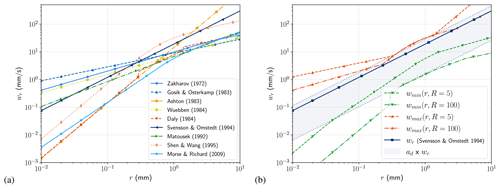Figure 4Comparison of frazil rise velocity models with R=10 (a) and the rise velocity envelope chosen for the uncertainty analysis (b) .

## 4.5 Summary of uncertain parameters

To conclude the uncertainty quantification, all the uncertain parameters, their bounds and PDFs are summarized in Table 2.

5 Uncertainty propagation and sensitivity analysis

In this section, we present the different Monte Carlo simulation cases considered for the uncertainty analysis, as synthesized in Table 3. Then we discuss the results of the uncertainty propagation and the sensitivity analysis obtained for both SSC and MSC models.

## 5.1 Propagation cases

Monte Carlo simulations are first carried out without seeding and gravitational removal (τs=0 and ad=0) for both SSC and MSC models, which correspond to cases 1 and 2, respectively. Seeding and gravitational removal processes are subsequently considered in cases 3 and 4. For each case, the set of uncertain parameters is described in Table 2. When not in X, parameters are considered deterministic with the following default values: ad=0, τs=0 m2 s−1, δT=e, ${r}_{\mathrm{min}}={\mathrm{10}}^{-\mathrm{5}}$ m and ${r}_{\mathrm{max}}={\mathrm{10}}^{-\mathrm{3}}$ m.

Table 3List of Monte Carlo simulations and their associated input random vectors X, sampling size and number of function calls. Seeding rate and buoyancy velocity are set to τs=0 and ad=0 in cases 1 and 2.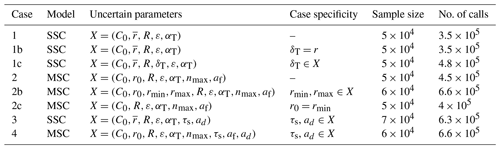Additional Monte Carlo simulations were carried out to examine the influence of specific parameters. For example, although δT=e is the preferred scaling and is chosen by default in all experiments, we also tested δT=r and ${\mathit{\delta }}_{\mathrm{T}}\in \left[e,r\right]$ in experiments 1b and 1c to investigate the impact on results. In experiments 2b and 2c, modifications of the MSC model uncertain parameters are also taken into account, in order to investigate the impact of the radius bounds rmin and rmax, as well as the alternate methods of initialization discussed in Sect. 4.2.

Statistical estimators are evaluated every 10 s of physical time, leading to nt=400. To cope with the computational cost of multi-class experiments, we used clusters to run all simulations in parallel. The computation time of 4.5×105 multi-class simulations with m=100 is ∼24 h with 960 processes. A total of 4 million simulations were carried out for uncertainty propagation.

## 5.2 Results without gravitational removal

By neglecting the seeding rate and gravitational removal source terms, the steady state corresponds to the constant frazil production rate that only depends on the heat sink ϕ, as shown in Sect. 2.5. As expected, the statistical estimator time series for temperature and frazil volume fraction, presented in Fig. 5 for cases 1 and 2, show a very narrow scatter of the output PDF at the start of simulation and at steady state. The results converge towards the two asymptotes (T0 and C respectively) of the mono-class ODE system: i.e., the constant cooling rate when t→0 (initial supercooling phase) and the constant frazil production rate when t→∞ (recovery phase). However, for both SSC and MSC models, the transition between the two asymptotes of the ODE system is spread out, and there is a significant difference in maximum supercooling between the 5th, 25th, 75th and 95th percentiles. For the median, a maximum supercooling of $T\left({t}_{\mathrm{c}}\right)\simeq -\mathrm{0.018}$C is reached at tc=180 s for the SSC model. The gap between the 5th and 95th percentile maximum supercooling is Δtc=890 s and Δθ=0.097C. Note that the envelope obtained with the standard deviation gives a poor description of the output since its PDF is not normal, as it can be seen from the $\stackrel{\mathrm{^}}{\mathit{\mu }}±\stackrel{\mathrm{^}}{\mathit{\sigma }}$ lines in the Fig. 5.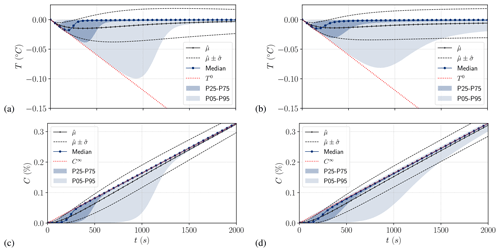Figure 5Uncertainty propagation results for SSC (case 1) for temperature (a) and frazil (c) and MSC (case 2) for temperature (b) and frazil (d): mean, standard deviation, median, and the 5th, 25th, 75th and 95th percentiles are computed for tk ($\mathrm{0}\le k\le {n}_{t}$).

Similar results were obtained with the MSC model, which recovers the same asymptote at steady state; however, there is a slight residual scatter at recovery. For case 2, we observed slightly less scatter at the maximum supercooling than with the SSC model, and the gap between the 5th and 95th percentile maximum supercooling was Δtc=820 s and Δθ=0.08C.

Time series of the first-order Sobol indices are presented in Fig. 6 (see Appendices E and F for details). In Appendix C, we also present the first- and total-order Sobol indices at times tc, tc and tf with 95 % confidence intervals, as well as the aggregated Sobol indices, computed via Eq. (B7). The time evolution of Sobol indices of temperature and frazil was similar for both the SSC and MSC with the exception of the initial concentration, which, as expected, had more of an impact on frazil volume fraction at the start of simulation.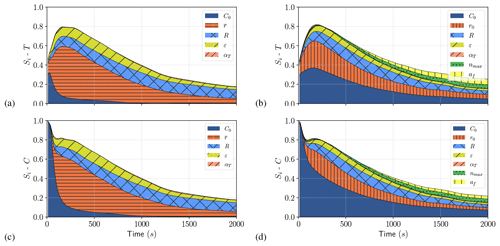Figure 6Time series of first-order Sobol indices (Si) for SSC (case 1) for temperature (a) and frazil (b) and MSC (case 2) for temperature (b) and frazil (d).

For the SSC model, the initial concentration plays an important role at the start of the simulation. However, its influence quickly decreases, and the most influential parameter becomes the radius, with a peak influence reached at the median value of the time of maximum supercooling. Thus, using the average radius as the calibration parameter of the SSC model seems to be the most relevant choice. The most influential parameters after radius are the diameter-to-thickness ratio and dissipation rate of turbulent kinetic energy. In experiments, the turbulent dissipation rate is often better known than the initial concentration or crystal shape. In natural water bodies, turbulent dissipation rate measurements are rare but turbulence models can be use to estimate these parameters. Therefore, one could then choose the initial frazil volume fraction or diameter-to-thickness ratio as a secondary calibration parameters.

For the MSC model, the most influential parameters prior to maximum supercooling are initial concentration (C0) and maximum initial radius (r0), with both control the initial distribution (and consequently the initial condition of the system). Using these initial distribution parameters to calibrate the transient phase until maximum supercooling seems to be the right approach. However, specifying a more accurate initial distribution (not necessarily uniform) by comparison with what can be observed in nature would be a welcome improvement, although this requires further research.

At the recovery, the parameters of secondary nucleation and flocculation processes (nmax and af), both impacting steady-state crystal distribution, become more influential. However, the hierarchy of parameters is less obvious than for the SSC model. In addition, we observed strong interactions between parameters when the model reaches steady state (see blank space on Fig. 6 and total Sobol indices in Appendix F), which might lead to difficulties in the calibration process. Aggregated Sobol indices summarized in Fig. 7 confirm the relative influence of each parameter over the whole duration of the simulation, taking into account both the transient phase and steady state.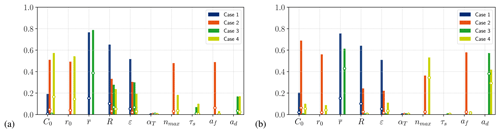Figure 7Aggregated first-order Sobol indices (dots) and aggregated total Sobol indices (bars) for temperature (a) and total frazil volume fraction (b) for cases 1, 2, 3 and 4.

While the approach adopted by – i.e., tweaking the values of nmax and af – was adequate to calibrate the frazil distribution in MSC models, the present sensitivity analysis shows that it might not be the best option to calibrate water temperature and frazil total volume fraction. Results suggests that more focus should be on the initial condition to calibrate supercooling, by modifying initial seeding like it was done by or by modifying the initial distribution itself. We therefore suggest calibrating the supercooling curve with the help of the initial distribution, along with secondary nucleation and flocculation parameters to calibrate the evolution of size distribution over time. Hopefully, recent observations of the transient evolution of frazil size distribution will provide the necessary data to carry out an optimal calibration of the identified parameters.

## 5.3 Influence of gravitational removal and seeding

Long-term evolution that does not take account of gravitational removal leads to infinite increase in frazil concentration as long as the cooling rate remains constant (see Eq. 15). This asymptotic behavior of the models has never been observed in experiment or in nature. observed a peak in the number of particles per image they recorded, located shortly after maximum supercooling, after which there was a small decrease in the number of particles and a stagnation at residual supercooling. Similar observations were also reported by and . The models in which only thermal growth is considered do not incorporate the required physics to properly reproduce what is observed. However by introducing gravitational removal, as shown in Sect. 2.5, the models converge towards a constant frazil volume fraction (see Eq. 16). In this section we analyze the results of the uncertainty propagation and sensitivity analysis for cases 3 and 4, which include the seeding rate and gravitational removal source terms.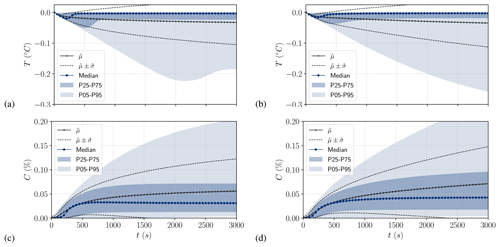Figure 8Uncertainty propagation results for SSC (case 3) for temperature (a) and frazil (c) and MSC (case 4) for temperature (b) and frazil (d): mean, standard deviation, median, and the 5th, 25th, 75th and 95th percentiles computed for tk ($\mathrm{0}\le k\le {n}_{t}$).

At steady state, the introduction of gravitational removal leads to wide scatter of both temperature and frazil as shown by the comparison of Figs. 8 and 5 (see Appendices G and H for details). This is caused by the variation of the steady-state frazil volume fraction, which depends on the heat flux ϕ and model parameters inherent in secondary nucleation, flocculation and rise velocity (nmax, af and ad). Significant supercooling values are observed as the water temperature (5th percentile) drops below −0.2C, which is really low compared to what can be observed in rivers. This is due to the highest values of the buoyancy velocity combined with low thermal growth rate. High gravitational removal withdraws a large amount of the frazil volume fraction from the water and thus limits the amount of latent heat release to water that would increase its temperature. No significant difference is observed in the output scatter between SSC and MSC models similarly to cases 1 and 2. However, minimum supercooling is not reached on the 5th percentile with the MSC as opposed to the SSC model. This is due to the dependency of the buoyancy velocity on the radius, which is not constant in the MSC model as opposed to the SSC model. This also causes the median frazil volume fraction at steady state to be slightly different in the two models.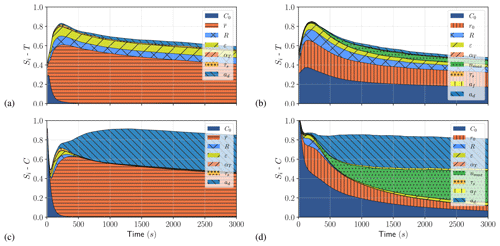Figure 9Time series of first-order Sobol indices (Si) for SSC (case 3) for temperature (a) and frazil (c) and MSC (case 4) for temperature (b) and frazil (d).

With the SSC model at recovery, the first-order Sobol indices on frazil volume fraction (SiC) in Fig. 9 show a major influence of the radius and the buoyancy coefficient (${S}_{\stackrel{\mathrm{‾}}{r}}=\mathrm{0.381}$ and ${S}_{{a}_{d}}=\mathrm{0.382}$, respectively at t=tf), the main parameters influencing the gravitational removal (see Eq. 15). For the MSC model, the most influential parameters at recovery are nmax and ad (${S}_{{n}_{\mathrm{max}}}=\mathrm{0.37}$ and ${S}_{{a}_{d}}=\mathrm{0.26}$, respectively at t=tf), which is consistent with Eqs. (27) and (29) of . The hierarchy of the most influential parameters is similar to cases 1 and 2 prior to maximum supercooling. However accurate modeling of the buoyancy velocity of frazil crystals is essential, as it has a very important influence on the long-term evolution of the system, and therefore merits particular attention.

## 5.4 Maximum supercooling scatter

The results discussed above were obtained with a cooling rate of −500 W m−3. Several cooling rates, ranging from −50 to −1000 W m−3, were tested with case (1) to assess variations in maximum supercooling predictions. We found that the higher the cooling rate, the greater the scatter of the predicted maximum supercooling temperature, as presented in Figs. 10 and 11. This is the opposite for the time until maximum supercooling peak, where the higher the cooling rate, the lower the scatter in supercooling time. These results conform to observations of and that the degree of supercooling increases with the heat sink rate while the time to supercooling decreases. Note that the gap between the 5th and 95th percentile maximum supercooling predictions is as much as Δtc=2540 s with $\mathit{\varphi }=-\mathrm{50}$ W m−3 and Δθ=0.142C with $\mathit{\varphi }=-\mathrm{1000}$ W m−3 as shown in Fig. 11. The dispersion both in time to supercooling and degree of supercooling is significant. This shows the importance of having a good quantification of input parameters to be able to predict the maximum supercooling point.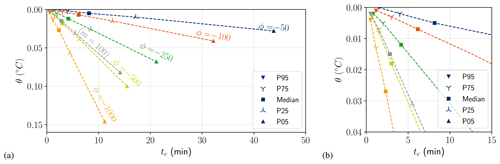Figure 10Maximum supercooling point scatter computed from median and 5th, 25th, 75th and 95th percentile time series at different cooling rates ($\mathit{\varphi }=-\mathrm{50}$, −100, −250, −500 and −1000 W m−3) for case 1 and with $\mathit{\varphi }=-\mathrm{500}$ W m−3 for case 2. Global view (a) and focus on initial time (b).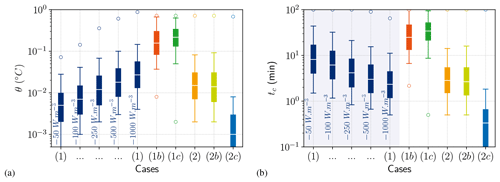Figure 11Maximum supercooling point scatter computed from median and 5th, 25th, 75th and 95th percentile time series at different cooling rates (−50, −100, −250, −500 and −1000 W m−3) for case 1; comparison between the choice of the length scale δT (cases 1b and 1c); and comparison between different initial conditions (cases 2b and 2c) with $\mathit{\varphi }=-\mathrm{500}$ W m−3. Maximum degree of supercooling (a) and maximum supercooling time (b). The white line is the median, the wide box is delimited by the 25th and 75th percentiles, the thin line is delimited by the 5th and 95th percentiles, and the round dots corresponds to minimum and maximum values.

Results obtained from different scalings of the thermal boundary layer are also shown in Fig. 11. A significant increase in scatter is observed for the δT=r compared to δT=λ scaling, consistent with the fact that thermal growth is clearly underestimated . With δT taken as an uncertain parameter with a log-uniform PDF within the bounds [min(λ), min(r)], the result is also more widely scattered by the same order of magnitude as with δT=r. The results highlight the significant influence of the choice of scaling for the boundary layer. Choice of scaling also explains inconsistencies in calibrated parameters in the literature.

Finally, let us discuss the results obtained with the MSC model, which need to be viewed from the standpoint of the way it is initialized. By taking the minimum and maximum radius as uncertain parameters (case 2b), we observe an increased gap between the 5th and 95th percentiles, and we have Δtc=930 s and Δθ=0.09C (see Fig. 11). The constant feed of first-class nuclei due to secondary nucleation accentuates the influence of the minimum radius parameter. This explains why the minimum radius has more influence on the results than the maximum radius as shown in Appendix D. Additionally, the volume growth rate being higher for small classes makes the initial distribution of concentration a determining choice. The more initial concentration is attributed to the smallest classes – the quicker the model reaches steady state, the less scatter is observed in maximum supercooling time. The extreme case is when the initial concentration is only applied to the first class (case 2c), when an astonishingly narrow scatter of results is observed. In fact, the transient evolution is so quick that the model almost instantaneously converges towards its steady state. The median maximum supercooling is only −0.001C and is reached in only 20 s. This confirms the sensitivity test carried out by , who suggested distributing initial concentration on one class. However, the results show that this type of initialization might not be the best option, as it almost totally does away with transient evolution of the model.

## 5.5 Limits and perspectives

The importance of the availability of quantitative field and laboratory data to support the uncertainty quantification of model parameters cannot be stressed enough. As we have shown, many model parameter uncertainties could not be characterized by means of direct data. We had to use expert knowledge as well as past numerical implementation . In part, this is because most physical processes, such as secondary nucleation or flocculation, are not directly measured but instead are inferred from frazil distribution observations. Moreover, some parameters, such as the initial condition, are dependent on the discretization method. Consequently, several parameter uncertainty bounds and PDFs should be refined. The modeling of dependency should also be taken into account in future work as many parameters, such as δT and ad, depend on other parameters (e.g., r and R). This would add a degree of complexity to the sensitivity analysis, but innovative methods to tackle parameter dependency could bring valuable new insight into the models.

In this paper, we considered a well-mixed water body and a simplified gravitational removal sink term. Spatial variations for temperature and frazil may result in different conclusions. A poorly mixed water body, where the cooling rate at higher layers is more severe than at the bottom, would cause a heterogeneity in the initial formation of frazil. Additionally, the meteorological seeding of frazil nuclei occurs mainly at the free surface, increasing the heterogeneity. Furthermore, as the rise velocity is higher for larger particles, the rise of frazil crystals and flocs yet again increases the heterogeneity of frazil on the vertical axis, altering the steady-state distributions, as it was shown in the study by . All these complex processes make the conclusions for a well-mixed body difficult to extrapolate to multidimensional cases. Further research therefore may be warranted into uncertainty quantification and sensitivity analyses of frazil ice models in non-well-mixed conditions with multidimensional models. The emergence of efficient APIs (application programming interface) in numerical tools such as TELEMAC-MASCARET , together with meta-modeling techniques that synthesize the essence of the multi-dimensional fields , could greatly assist such an undertaking.

Viewed from a different perspective, one may use the same probabilistic framework to compare modeling approaches for each process. This could be done by focusing on the volume fraction of each class to obtain a good picture of how frazil is distributed over radius. In the same way that we considered time series of total frazil volume fraction as output, one could easily transpose the analysis to multi-dimensional class volume fraction as output. Uncertainty propagation could then allow for the characterization of PDFs associated with each class, and sensitivity analysis would shed light on the properties of each model and how they affect frazil distributions. This could be a valuable tool for inferring new laws of secondary nucleation or flocculation by comparison to the observed evolution of frazil distributions.

6 Summary and conclusions

In this paper, two mathematical models for predicting of the evolution of frazil ice volume fraction and temperature have been studied. We developed a multiple-size-class MSC model, relying on the radial space discretization of frazil ice dynamic equations introduced by Daly (1984), which includes processes such as thermal growth, secondary nucleation, flocculation, seeding and gravitational removal. A simplified single-size-class SSC model, including only thermal growth, seeding and gravitational removal, was also developed for comparison. Properties of the two models, such as their steady states, were highlighted, and details were provided for their numerical resolution. We found that, for proper resolution of the transient phase with the MSC model, a class number of about 100 is necessary for convergence of the results, which corroborates the observations by . Uncertainties in both models were then studied within a probabilistic framework. Aided by recent experimental and field studies of frazil ice, and also by numerical studies carried out in the recent years, the uncertainty of the main parameters of the frazil ice models was quantified. Various Monte Carlo experiments were considered to propagate the uncertainties. Time-dependent statistical estimates of the models' outputs (temperature and frazil volume fraction) were then analyzed, and a sensitivity analysis was carried out by means of a variance decomposition method.

Given the uncertainty bounds defined in the present study, SSC and MSC models yield very similar results for the prediction of water temperature and total frazil volume fraction. In the absence of gravitational removal, we have shown that the uncertainties have a great impact on the maximum supercooling and recovery time but scarcely any impact on the steady state, which is governed only by cooling rate. The more detailed physics of the multi-class model, although providing valuable new information on size distribution of the crystals, does not make it possible to obtain a more reliable estimate of water temperature and total frazil volume fraction in the transient phase. The development of MSC models raises the possibility that uncertainty may be removed from choosing a mean radius. We have shown, however, that scatter is similar somehow in both models and derives from new uncertain parameters inherent in radial space discretization. Note that, in many numerical tools, modeling frazil distribution requires the resolution of multiple advection–diffusion equations. Given the number of classes required for a model convergence, one can easily grasp the high numerical cost of using the MSC model for large-scale, multi-dimensional applications. This makes the SSC model a suitable candidate for multi-dimensional frazil ice modeling, and the present study shows that it is still a very good compromise between uncertainty and model complexity. However, it should be noted that such uncertainty in the MSC model could be overcome in future laboratory experiments by a better estimation of the initial crystal size distribution.

The sensitivity analysis allowed us to address with confidence the choice of calibration parameters. Relying on first- and total-order Sobol indices, we quantified the relative influence of each uncertain parameter on the output distribution, for both SSC and MSC models, and proposed a selection of parameters to be used for calibration. For the SSC model, the most influential factor for both temperature and frazil is the mean radius. Initial concentration played a secondary role although it was initially identified as a predominant factor. We therefore suggest using the average radius as the main calibration parameter. The turbulent dissipation rate also plays a major role and as such should be specified with care. As it can be estimated via turbulence models, we suggest using initial concentration and diameter-to-thickness ratio as secondary calibration parameters. With the MSC model, we showed that the dispersion is somehow similar to what we observed for the SSC model but originates from new uncertain parameters. Thus, the most influential parameters on the transient phase are the parameters specific to the initial condition. However, once the steady state was reached, we observed an increasing influence of the secondary nucleation and flocculation parameters. The long-term evolution of the system also showed increasing interactions between parameters. This could be explained by the balance in the physical processes involved in class interactions, and further investigation using class volume fraction as model outputs would help. When gravitational removal was introduced in the models, the stationary state was modified and the concentration converged towards a finite limit instead of diverging. In the case of the SSC model, the asymptotic limit is a function of the ratio between the gravitational removal term and the heat flux, while in the case of the MSC model, the stationary state is also a function of the steady-state radius distribution (which depends on the balance between secondary nucleation, flocculation and gravitational removal). Our study, confirming previous asymptotic analyses, showed that both secondary nucleation and gravitational removal parameters are the most influential on total frazil volume fraction. The buoyant rise velocity, the uncertainty of which was rarely taken into account in previous modeling studies, should therefore be one of the main foci of future efforts to calibrate frazil ice models. Contrary to frazil, water temperature was mostly influenced by the initial condition, even at steady state. This should impel us to use both water temperature and frazil volume fraction measurements to calibrate the models. Fortunately, recent laboratory and field studies , particularly on the evolution of frazil distributions over time, offer precious data that can assist in developing appropriate calibration of the models. In this regard, using optimal calibration techniques that allow consideration of both modeling and data uncertainties would be a natural and complementary extension of the present study.

Appendix A: Semi-implicit theta scheme matrices

In this section, the semi-implicit time discretization of Eqs. (7) and (12) is described. Let us denote ${t}_{k}={t}_{\mathrm{0}}+k\mathrm{\Delta }t$ the time at iteration k, t0 the initial time and ck=c(tk). The semi-implicit time scheme consists in posing ${c}_{i}=\mathit{\theta }{c}_{i}^{k+\mathrm{1}}+\left(\mathrm{1}-\mathit{\theta }\right){c}_{i}^{k}$ in the right-hand side of Eq. (7). Choosing θ=0 leads to a fully explicit time scheme, while choosing θ=1 is equivalent to an implicit scheme on c. The non-linear terms are treated semi-implicitly, i.e., Gi=Gi(tk) in order to retrieve a linear system of the form $\mathbf{A}\left[{c}_{\mathrm{1}}^{k+\mathrm{1}}$, …, ${c}_{m}^{k+\mathrm{1}}{\right]}^{T}=\mathbf{B}$ in which A and B are two matrices defined below. The temperature balance equation is then solved with a forward Euler time scheme.

The matrix system reads $\mathbf{A}\left[{c}_{\mathrm{1}}^{k+\mathrm{1}}$, …, ${c}_{m}^{k+\mathrm{1}}{\right]}^{T}=\mathbf{B}⇔$

$\begin{array}{}\text{(A1)}& \begin{array}{rl}& \left(\begin{array}{cccccccc}& {a}_{\mathrm{11}}& {a}_{\mathrm{12}}& {a}_{\mathrm{13}}& & \mathrm{\dots }& & {a}_{\mathrm{1}m}\\ & {a}_{\mathrm{21}}& {a}_{\mathrm{22}}& {a}_{\mathrm{23}}& \mathrm{0}& \mathrm{\dots }& & \mathrm{0}\\ & \mathrm{0}& \mathrm{\ddots }& \mathrm{\ddots }& \mathrm{\ddots }& & & \\ & & & {a}_{ii-\mathrm{1}}& {a}_{ii}& {a}_{ii+\mathrm{1}}& & \mathrm{⋮}\\ & \mathrm{⋮}& & & \mathrm{\ddots }& \mathrm{\ddots }& \mathrm{\ddots }& \mathrm{0}\\ & & & & & & & {a}_{m-\mathrm{1}m}\\ & \mathrm{0}& & \mathrm{\dots }& & \mathrm{0}& {a}_{mm-\mathrm{1}}& {a}_{mm}\end{array}\right)\\ & \left(\begin{array}{cc}& {c}_{\mathrm{1}}^{k+\mathrm{1}}\\ & \mathrm{⋮}\\ & \\ & {c}_{i}^{k+\mathrm{1}}\\ & \mathrm{⋮}\\ & \\ & {c}_{m}^{k+\mathrm{1}}\end{array}\right)=\left(\begin{array}{cc}& {b}_{\mathrm{1}}\\ & \mathrm{⋮}\\ & \\ & {b}_{i}\\ & \mathrm{⋮}\\ & \\ & {b}_{N}\end{array}\right),\end{array}\end{array}$

in which the diagonal terms are defined as

$\begin{array}{ll}& {a}_{\mathrm{11}}=\mathrm{1}-\mathit{\theta }\mathrm{\Delta }t\left({V}_{\mathrm{1}}\left({\mathrm{\Lambda }}_{\mathrm{1}}-{\mathrm{\Gamma }}_{\mathrm{1}}\right)-{\mathit{\beta }}_{\mathrm{1}}-{\mathit{\gamma }}_{\mathrm{1}}\right),\\ & {a}_{ii}=\mathrm{1}-\mathit{\theta }\mathrm{\Delta }t\left({V}_{i}\left({\mathrm{\Lambda }}_{i}-{\mathrm{\Gamma }}_{i}\right)-{\mathit{\beta }}_{i}-{\mathit{\gamma }}_{i}-\mathit{\zeta }{\mathit{\alpha }}_{i}\right)\\ & \mathrm{for}\phantom{\rule{0.25em}{0ex}}\mathrm{2}\le i\le m-\mathrm{1},\\ & {a}_{mm}=\mathrm{1}-\mathit{\theta }\mathrm{\Delta }t\left({V}_{m}{\mathrm{\Lambda }}_{m}-{\mathit{\gamma }}_{m}-\mathit{\zeta }{\mathit{\alpha }}_{m}\right),\end{array}$

the lower off-diagonal terms are defined as

${a}_{ii-\mathrm{1}}=-\mathit{\theta }\mathrm{\Delta }t\left({V}_{i}{\mathrm{\Gamma }}_{i-\mathrm{1}}+{\mathit{\beta }}_{i-\mathrm{1}}\right)\phantom{\rule{0.25em}{0ex}}\mathrm{for}\phantom{\rule{0.25em}{0ex}}\mathrm{2}\le i\le m,$

the upper off-diagonal terms are defined as

$\begin{array}{ll}& {a}_{\mathrm{1}i}=-\mathit{\theta }\mathrm{\Delta }t{\mathit{\alpha }}_{i}\phantom{\rule{0.25em}{0ex}}\mathrm{for}\phantom{\rule{0.25em}{0ex}}\mathrm{2}\le i\le m,\\ & {a}_{ii+\mathrm{1}}=\mathit{\theta }\mathrm{\Delta }t{V}_{i}{\mathrm{\Lambda }}_{i+\mathrm{1}}\phantom{\rule{0.25em}{0ex}}\mathrm{for}\phantom{\rule{0.25em}{0ex}}\mathrm{2}\le i\le m-\mathrm{1},\end{array}$

and the matrix B is defined as

Appendix B: Sensitivity analysis using Sobol indices

For a given set of independent input parameters, the ANOVA (analyses of variance) decomposition allows us to compute the variance of output $\mathbit{Y}=g\left(\mathbit{X},\mathbit{d}\right)$ for each time step tk ($\mathrm{1}\le k\le {n}_{t}$) as

$\begin{array}{}\text{(B1)}& \begin{array}{rl}\mathrm{Var}\left[{Y}^{k}\right]& =\sum _{i=\mathrm{1}}^{{n}_{X}}{V}_{i}\left({Y}^{k}\right)+\sum _{i

where

$\begin{array}{ll}& {V}_{i}\left({Y}^{k}\right)=\mathrm{Var}\left[\mathbb{E}\left[{Y}^{k}|{X}^{i}\right]\right]\\ & {V}_{ij}\left({Y}^{k}\right)=\mathrm{Var}\left[\mathbb{E}\left[{Y}^{k}|{X}^{i},{X}^{j}\right]-\mathbb{E}\left[{Y}^{k}|{X}^{i}\right]-\mathbb{E}\left[{Y}^{k}|{X}^{j}\right]\right]\\ & =\mathrm{Var}\left[\mathbb{E}\left[{Y}^{k}|{X}^{i},{X}^{j}\right]\right]-{V}_{i}\left({Y}^{k}\right)-{V}_{j}\left({Y}^{k}\right),\end{array}$

in which 𝔼[Yk|Xi] represents the conditional expectation of Yk with the condition that Xi remains constant.

To evaluate the influence of each input parameter, the so-called Sobol indices are used (Sobol2001). The first- and second-order Sobol indices are defined as follows, for $k\in \mathit{\left\{}\mathrm{1},\phantom{\rule{0.125em}{0ex}}\mathrm{\dots },\phantom{\rule{0.125em}{0ex}}{n}_{t}\mathit{\right\}}$ and $i\in \mathit{\left\{}\mathrm{1},\phantom{\rule{0.125em}{0ex}}\mathrm{\dots },\phantom{\rule{0.125em}{0ex}}{n}_{X}\mathit{\right\}}$:

$\begin{array}{}\text{(B2)}& \begin{array}{r}{S}_{i}^{k}=\frac{{V}_{i}\left({Y}^{k}\right)}{\mathrm{Var}\left[{Y}^{k}\right]},\\ {S}_{ij}^{k}=\frac{{V}_{ij}\left({Y}^{k}\right)}{\mathrm{Var}\left[{Y}^{k}\right]}.\end{array}\end{array}$

The first-order Sobol index ${S}_{i}^{k}$ indicates the part of output variance explained by a single parameter Xi without interactions, whereas second-order indices ${S}_{ij}^{k}$ quantify the part of variance of the output explained by the interaction between two inputs Xi and Xj. The number of second-order indices is given by $\left(\underset{{n}_{X}}{\mathrm{2}}\right)={n}_{X}\left({n}_{X}-\mathrm{1}\right)/\mathrm{2}$. When the number of input parameters is too large, it may be difficult to estimate second-order indices. In that case, we only estimate first-order and total indices. Total indices quantify the part of variance of the output explained by an input and its interactions with all the other inputs parameters. Total Sobol indices are defined as follows, for $k\in \mathit{\left\{}\mathrm{1},\phantom{\rule{0.125em}{0ex}}\mathrm{\dots },\phantom{\rule{0.125em}{0ex}}{n}_{t}\mathit{\right\}}$ and $i\in \mathit{\left\{}\mathrm{1},\phantom{\rule{0.125em}{0ex}}\mathrm{\dots },\phantom{\rule{0.125em}{0ex}}{n}_{X}\mathit{\right\}}e$:

$\begin{array}{}\text{(B3)}& \begin{array}{rl}S{T}_{i}^{k}& ={S}_{i}^{k}+\sum _{i\ne j}{S}_{ij}^{k}+\sum _{i\ne j,l\ne i,j\le l}{S}_{ijl}^{k}\\ & +\phantom{\rule{0.125em}{0ex}}\mathrm{\dots }\phantom{\rule{0.125em}{0ex}}=\frac{V{T}_{i}\left({Y}^{k}\right)}{\mathrm{Var}\left[{Y}^{k}\right]}=\mathrm{1}-\frac{{V}_{-i}\left({Y}^{k}\right)}{\mathrm{Var}\left[{Y}^{k}\right]},\end{array}\end{array}$

where ${V}_{-i}\left({Y}^{k}\right)=\mathrm{Var}\left[\mathbb{E}\left[{Y}^{k}|{X}^{\mathrm{1}},\phantom{\rule{0.125em}{0ex}}\mathrm{\dots },\phantom{\rule{0.125em}{0ex}}{X}^{i-\mathrm{1}},{X}^{i+\mathrm{1}},\phantom{\rule{0.125em}{0ex}}\mathrm{\dots },\phantom{\rule{0.125em}{0ex}}{X}^{{n}_{x}}\right]\right]$.

To compute Sobol indices, a modified version of the method proposed by is used , in which two independent samples of size N, denoted A and B, are generated. Both can be written as matrices (see Eq. B4) in which each line is a realization of the random vector X. A third matrix, denoted Ci, is then created by replacing only the column i of the matrix A by the column i of the matrix B (see Eq. B4):

$\begin{array}{}\text{(B4)}& \begin{array}{rl}\mathbf{A}& =\left(\begin{array}{cccc}{x}_{\mathrm{1}}^{A,\mathrm{1}}& {x}_{\mathrm{2}}^{A,\mathrm{1}}& \mathrm{\dots }& {x}_{{n}_{X}}^{A,\mathrm{1}}\\ {x}_{\mathrm{1}}^{A,\mathrm{2}}& {x}_{\mathrm{2}}^{A,\mathrm{2}}& \mathrm{\dots }& {x}_{{n}_{X}}^{A,\mathrm{2}}\\ \mathrm{⋮}& \mathrm{⋮}& \mathrm{\ddots }& \mathrm{⋮}\\ {x}_{\mathrm{1}}^{A,N}& {x}_{\mathrm{2}}^{A,N}& \mathrm{\dots }& {x}_{{n}_{X}}^{A,N}\end{array}\right),{\mathbf{C}}^{i}\\ & =\left(\begin{array}{ccccc}{x}_{\mathrm{1}}^{A,\mathrm{1}}& \mathrm{\dots }& {x}_{i}^{B,\mathrm{1}}& \mathrm{\dots }& {x}_{{n}_{X}}^{A,\mathrm{1}}\\ {x}_{\mathrm{1}}^{A,\mathrm{2}}& \mathrm{\dots }& {x}_{i}^{B,\mathrm{2}}& \mathrm{\dots }& {x}_{{n}_{X}}^{A,\mathrm{2}}\\ \mathrm{⋮}& & \mathrm{⋮}& \mathrm{\ddots }& \mathrm{⋮}\\ {x}_{\mathrm{1}}^{A,N}& \mathrm{\dots }& {x}_{i}^{B,N}& \mathrm{\dots }& {x}_{{n}_{X}}^{A,N}\end{array}\right).\end{array}\end{array}$

First-order and total Sobol indices are computed using estimations of Vi(Yk) and Vi(Yk) computed using samples A, B, and Ci and denoted ${\stackrel{\mathrm{^}}{V}}_{i}\left({Y}^{k}\right)$ and ${\stackrel{\mathrm{^}}{V}}_{-i}\left({Y}^{k}\right)$ respectively. These estimations are defined as follows:

$\begin{array}{}\text{(B5)}& \begin{array}{rl}{\stackrel{\mathrm{^}}{V}}_{i}\left({Y}^{k}\right)& =\frac{\mathrm{1}}{N-\mathrm{1}}\sum _{j=\mathrm{1}}^{N}\stackrel{\mathrm{̃}}{g}\left({\mathbf{B}}_{j}\right)\stackrel{\mathrm{̃}}{g}\left({\mathbf{C}}_{j}^{i}\right)\\ & -\left(\frac{\mathrm{1}}{N}\sum _{j=\mathrm{1}}^{N}\stackrel{\mathrm{̃}}{g}\left({\mathbf{A}}_{j}\right)\right)\left(\frac{\mathrm{1}}{N}\sum _{j=\mathrm{1}}^{N}\stackrel{\mathrm{̃}}{g}\left({\mathbf{B}}_{j}\right)\right),\\ & {\stackrel{\mathrm{^}}{V}}_{-i}\left({Y}^{k}\right)=\frac{\mathrm{1}}{N-\mathrm{1}}\sum _{j=\mathrm{1}}^{N}\stackrel{\mathrm{̃}}{g}\left({\mathbf{A}}_{j}\right)\stackrel{\mathrm{̃}}{g}\left({\mathbf{C}}_{j}^{i}\right)\\ & -\left(\frac{\mathrm{1}}{N}\sum _{j=\mathrm{1}}^{N}\stackrel{\mathrm{̃}}{g}\left({\mathbf{A}}_{j}\right)\right)\left(\frac{\mathrm{1}}{N}\sum _{j=\mathrm{1}}^{N}\stackrel{\mathrm{̃}}{g}\left({\mathbf{B}}_{j}\right)\right),\end{array}\end{array}$

where $\stackrel{\mathrm{̃}}{g}$ is the centered model defined by $\stackrel{\mathrm{̃}}{g}=g-\stackrel{\mathrm{‾}}{g}$ in which $\stackrel{\mathrm{‾}}{g}$ is the sample mean of the combined output samples g(A) and g(B). To compute the second-order Sobol indices, an additional matrix is used, denoted ${{\mathbf{C}}^{\prime }}^{j}$, which is created by replacing only the column i of the matrix B by the column i of the matrix A. Then the estimation ${\stackrel{\mathrm{^}}{V}}_{ij}\left({Y}^{k}\right)$ is computed as

$\begin{array}{}\text{(B6)}& \begin{array}{rl}{\stackrel{\mathrm{^}}{V}}_{ij}\left({Y}^{k}\right)& =\frac{\mathrm{1}}{N-\mathrm{1}}\sum _{m=\mathrm{1}}^{N}\stackrel{\mathrm{̃}}{g}\left({\mathbf{C}}_{m}^{i}\right)\stackrel{\mathrm{̃}}{g}\left({{\mathbf{C}}^{\prime }}_{m}^{j}\right)\\ & -\frac{\mathrm{1}}{N}\sum _{m=\mathrm{1}}^{N}\stackrel{\mathrm{̃}}{g}\left({\mathbf{A}}_{m}\right)\stackrel{\mathrm{̃}}{g}\left({\mathbf{B}}_{m}\right)-{\stackrel{\mathrm{^}}{V}}_{i}\left({Y}^{k}\right)-{\stackrel{\mathrm{^}}{V}}_{j}\left({Y}^{k}\right).\end{array}\end{array}$

For a sample size N, estimation of the first-order and total Sobol indices requires $\left({n}_{X}+\mathrm{2}\right)×N$ simulations.

For multivariate outputs, the indices can be aggregated as proposed by . The aggregated first-order and total Sobol indices are defined as

$\begin{array}{}\text{(B7)}& \begin{array}{r}A{S}_{i}=\frac{\sum _{k=\mathrm{1}}^{{n}_{t}}{V}_{i}\left({Y}^{k}\right)}{\sum _{k=\mathrm{1}}^{{n}_{t}}\mathrm{Var}\left[{Y}^{k}\right]},\\ AS{T}_{i}=\frac{\sum _{k=\mathrm{1}}^{{n}_{t}}V{T}_{i}\left({Y}^{k}\right)}{\sum _{k=\mathrm{1}}^{{n}_{t}}\mathrm{Var}\left[{Y}^{k}\right]}.\end{array}\end{array}$

This means that Sobol indices ${S}_{i}^{k}$ and $S{T}_{i}^{k}$ quantify the influence of Xi on the variance of Y at time tk, while the aggregated indices ASi and ASTi quantify the influence of Xi over the whole time series of Y.

Appendix C: Sturdiness of statistical estimators

Convergence of statistical estimators is addressed by running several Monte Carlo simulations with increasing sampling size. An example of the convergence of mean and standard deviation at different times is given in Fig. C1 for case 1. Similarly, testing of Sobol index convergence is shown in Fig. C2 for case 1. Note that the 5th and 95th confidence intervals are systematically computed by a bootstrap method and plotted in all Sobol index figures.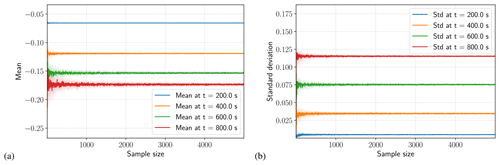Figure C1Mean and standard deviation convergence for case 1 for temperature (a) and frazil (b).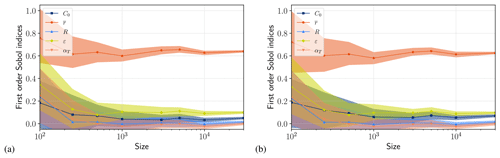Figure C2First-order Sobol index convergence for case 1 for temperature (a) and frazil (b).

Appendix D: Sobol indices and aggregated Sobol indices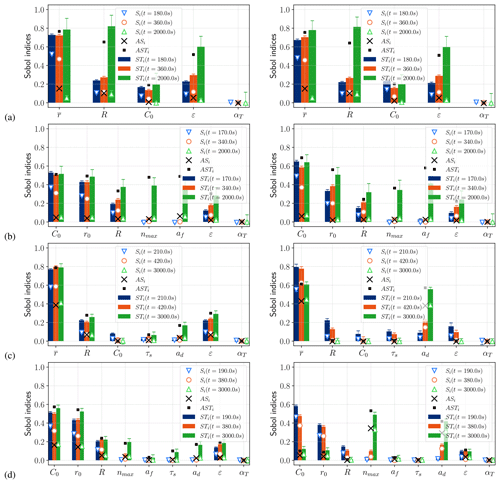Figure D1First-order and total-order Sobol indices at times tmin, 2tmin and tf and aggregated Sobol indices for temperature (left panels) and frazil (right panels) for case 1 (a), 2 (b), 3 (c) and 4 (d) from top to bottom.

Appendix E: Aggregated Sobol indices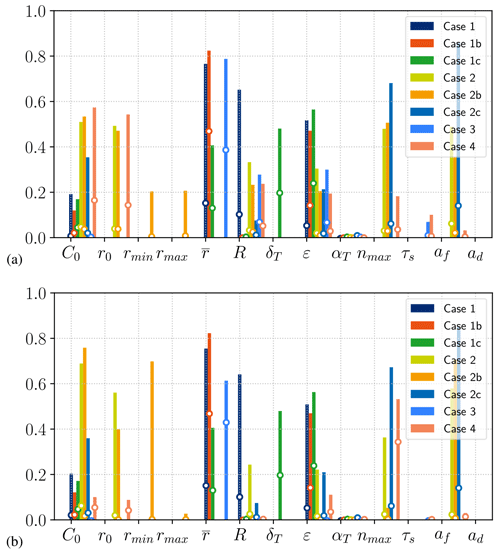Figure E1Aggregated first-order Sobol indices (dots) and aggregated total Sobol indices (bars) for temperature (a) and total frazil volume fraction (b) for case 1, 1b, 1c, 2, 2b, 2c, 3 and 4.

Appendix F: Results of case 1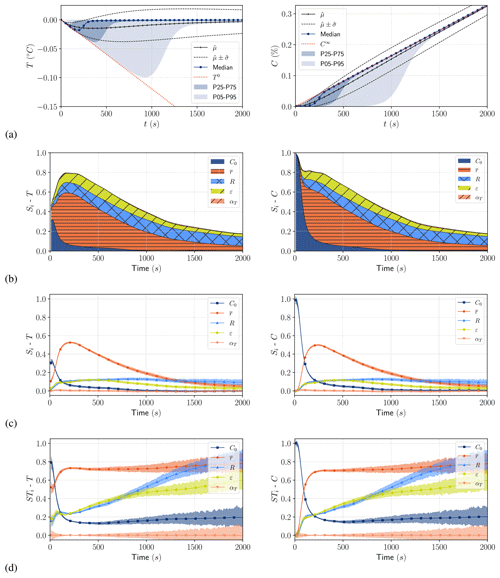Figure F1Results of case 1. From top to bottom: uncertainty propagation result (a), first-order Sobol indices (b), first-order Sobol indices with 95 % confidence intervals (c), and total-order Sobol indices with 95 % confidence intervals (d) for temperature (left panels) and frazil (right panels).

Appendix G: Results of case 2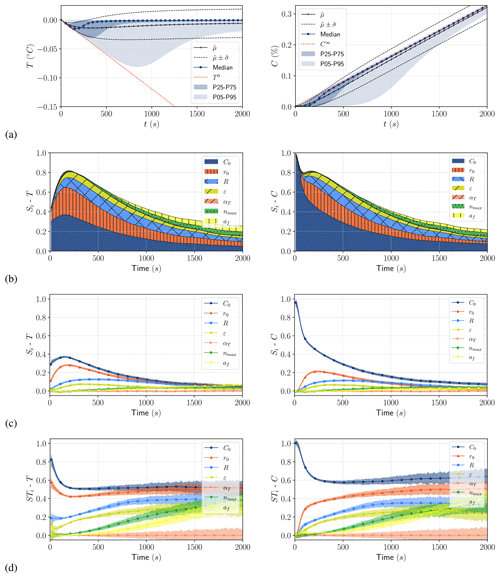Figure G1Results of case 2. From top to bottom: uncertainty propagation result (a), first-order Sobol indices (b), first-order Sobol indices with 95 % confidence intervals (c), and total-order Sobol indices with 95 % confidence intervals (d) for temperature (left panels) and frazil (right panels).

Appendix H: Results of case 3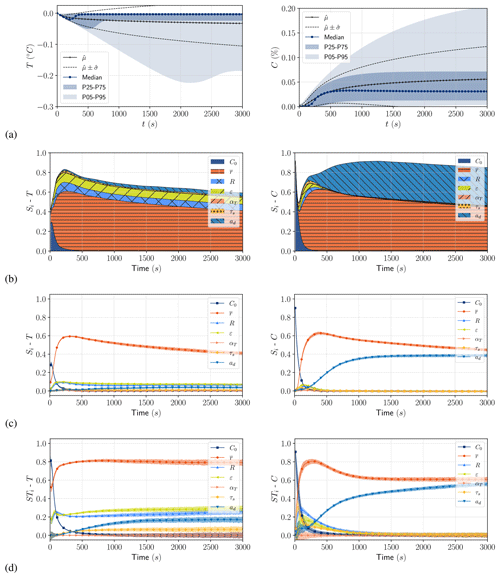Figure H1Results of case 3. From top to bottom: uncertainty propagation result (a), first-order Sobol indices (b), first-order Sobol indices with 95 % confidence intervals (c), and total-order Sobol indices with 95 % confidence intervals (d) for temperature (left panels) and frazil (right panels).

Appendix I: Results of case 4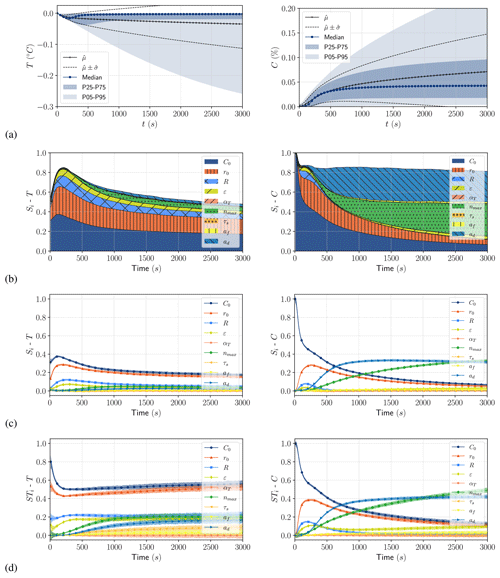Figure I1Results of case 4. From top to bottom: uncertainty propagation result (a), first-order Sobol indices (b), first-order Sobol indices with 95 % confidence intervals (c), and total-order Sobol indices with 95 % confidence intervals (d) for temperature (left panels) and frazil (right panels).

Code availability

Frazil ice models developed in this paper have been partially integrated within the TELEMAC-MASCARET open-source suite of solvers (see http://www.opentelemac.org, ) by the authors. The uncertainty analysis was carried out using OpenTURNS (Open source initiative for the Treatment of Uncertainties, Risks'N Statistics) , which can be found at http://openturns.github.io/openturns/latest/index.html .

Data availability

Author contributions

FS, CG and RSM conceptualized the study. FS conducted the analyses, interpreted the results and wrote the manuscript with input from CG and RSM.

Competing interests

The contact author has declared that none of the authors has any competing interests.

Disclaimer

Publisher's note: Copernicus Publications remains neutral with regard to jurisdictional claims in published maps and institutional affiliations.

Acknowledgements

The authors would like to thank Mark Loewen and David W. Rees Jones, whose comments and suggestions helped improve the scientific quality of this paper. The authors would also like to extend special thanks to Michael Baudin for his reading and discussion, which enriched this paper. The authors gratefully acknowledge contributions from the OpenTURNS open-source community.

Review statement

This paper was edited by Christian Haas and reviewed by Mark Loewen and David Rees Jones.

References

Arakawa, K.: Studies on the Freezing of Water (II) Formation of disc crystals, J. Facul. Sci. Hokkaido Univers., 4, 311–339, 1954. a, b, c

Ashton, G.: Frazil ice, in: Theory of dispersed multiphase flow, Elsevier, 271–289, https://doi.org/10.1016/B978-0-12-493120-6.50017-9, 1983. a

Barrette, P. D.: A tabulated review of 83 laboratory studies on frazil ice, in: IAHR International Symposium on Ice, 14–18 June 2020, Trondheim, Norway, https://www.iahr.org/library/infor?pid=8546 (last access: 11 April 2023), 2020. a

Barrette, P. D.: Understanding frazil ice: The contribution of laboratory studies, Cold Reg. Sci. Technol., 189, 103334, https://doi.org/10.1016/j.coldregions.2021.103334, 2021. a

Baudin, M., Boumhaout, K., Delage, T., Iooss, B., and Martinez, J.-M.: Numerical stability of Sobol' indices estimation formula, in: Proceedings of the 8th International Conference on Sensitivity Analysis of Model Output (SAMO 2016), 30 November–3 December 2016, Le Tampon, Réunion Island, France, https://www.gdr-mascotnum.fr/media/samo2016-sobol_vf.pdf (last access: 11 April 2023), 2016a. a

Baudin, M., Dutfoy, A., Iooss, B., and Popelin, A.-L.: OpenTURNS: An Industrial Software for Uncertainty Quantification in Simulation, Springer International Publishing, 1–38, https://doi.org/10.1007/978-3-319-11259-6_64-1, 2016b. a, b

Bombosch, A. and Jenkins, A.: Modeling the formation and deposition of frazil ice beneath Filchner-Ronne Ice Shelf, J. Geophys. Res.-Oceans, 100, 6983–6992, 1995. a

Carstens, T.: Experiments with supercooling and ice formation in flowing water, Geofys. Publ. Norway, 26, 3–18, 1966. a, b, c, d, e, f, g, h, i

Clark, S. and Doering, J.: Laboratory Experiments on Frazil-Size Characteristics in a Counterrotating Flume, J. Hydraul. Eng., 132, 94–101, https://doi.org/10.1061/(ASCE)0733-9429(2006)132:1(94), 2006. a, b, c, d, e

Clark, S. and Doering, J.: Frazil flocculation and secondary nucleation in a counter-rotating flume, Cold Reg. Sci. Technol., 55, 221–229, https://doi.org/10.1016/j.coldregions.2008.04.002, 2009. a

Clark, S. and Doering, J. C.: A laboratory study of frazil ice size distributions, in: Proc. 17th Int. Symp. on Ice, 21–25 June 2004, Saint Petersburg, Russia, 291–297, https://www.iahr.org/index/committe/14 (last access: 12 April 2023), 2004. a, b, c, d, e, f

Daly, S. F.: Frazil Ice Dynamics, Technical Report 84-1, US Army Cold Regions Research and Engineering Laboratory, Hanover, New Hampshire, https://erdc-library.erdc.dren.mil/jspui/bitstream/11681/2659/1/CRREL-Monograph-84-1.pdf (last access: 11 April 2023), 1984. a, b, c, d, e, f, g, h, i, j, k

Daly, S. F.: Frazil Ice Blockage of Intake Trash Racks, Cold Regions Technical Digest No. 91-1, Tech. rep., US Army Corps of Engineers, https://erdc-library.erdc.dren.mil/jspui/bitstream/11681/2677/1/CRREL-TD-91-1.pdf (last access: 11 April 2023), 1991. a

Daly, S. F.: Report on Frazil Ice, Tech. rep., Special Report 94-23, International Association for Hydraulic Research, Working Group on Thermal Regimes, https://erdc-library.erdc.dren.mil/jspui/bitstream/11681/12325/1/SR-94-23.pdf (last access: 11 April 2023), 1994. a, b, c, d, e, f, g, h

Daly, S. F.: Frazil Ice Blockage of Water Intakes in the Great Lakes, J. Hydraul. Eng., 2006. a

Daly, S. F. and Colbeck, S. C.: Frazil ice measurements in CRREL's flume facility, in: IAHR Symposium on Ice 1986 – Proceedings, vol. 1, 7–31 August 1986, Iowa, USA, 427–438, https://www.iahr.org/index/committe/14 (last access: 11 April 2023), 1986. a, b, c

Doering, J. C. and Morris, M. P.: River Ice Engineering/Ingénierie des glaces fluviales A digital image processing system to characterize frazil ice, Can. J. Civ. Eng., 30, 1–10, https://doi.org/10.1139/l02-028, 2003. a

Ettema, R., Karim, M., and Kennedy, J.: Laboratory experiments on frazil ice growth in supercooled water, Cold Reg. Sci. Technol., 10, 43–58, https://doi.org/10.1016/0165-232X(84)90032-6, 1984. a

Frazer, E. K., Langhorne, P. J., Leonard, G. H., Robinson, N. J., and Schumayer, D.: Observations of the size distribution of frazil ice in an Ice Shelf Water plume, Geophys. Res. Lett., 47, e2020GL090498, https://doi.org/10.1029/2020GL090498, 2020. a, b

Gamboa, F., Janon, A., Klein, T., and Lagnoux, A.: Sensitivity analysis for multidimensional and functional outputs, Elect. J. Stat., 8, 575–603, 2014. a, b

Ghobrial, T. R., Loewen, M. R., and Hicks, F.: Laboratory calibration of upward looking sonars for suspended frazil ice concentration, Cold Reg. Sci. Technol., 70, 19–31, https://doi.org/10.1016/j.coldregions.2011.08.010, 2012. a, b, c

Ghobrial, T. R., Loewen, M. R., and Hicks, F. E.: Characterizing suspended frazil ice in rivers using upward looking sonars, Cold Reg. Sci. Technol., 86, 113–126, https://doi.org/10.1016/j.coldregions.2012.10.002, 2013. a

Goeury, C., Audouin, Y., and Zaoui, F.: Interoperability and computational framework for simulating open channel hydraulics: Application to sensitivity analysis and calibration of Gironde Estuary model, Environ. Model. Softw., 148, 105243, https://doi.org/10.1016/j.envsoft.2021.105243, 2022. a

Gosink, J. P. and Osterkamp, T. E.: Measurements and Analyses of Velocity Profiles and Frazil Ice-Crystal Rise Velocities During Periods of Frazil-Ice Formation in Rivers, Ann. Glaciol., 4, 79–84, https://doi.org/10.3189/S0260305500005279, 1983. a, b

Hammar, L. and Shen, H. T.: A mathematical model for frazil ice evolution and transport in channels, in: Proc. 6th Workshop on the Hydraulics of River Ice, 23–15 October 1991, Ottawa, 201–206, http://www.cripe.ca/docs/proceedings/06/All_Proceedings.pdf (last access: 11 April 2023), 1991. a, b

Hammar, L. and Shen, H. T.: Frazil evolution in channels, J. Hydraul. Res., 33, 291–306, https://doi.org/10.1080/00221689509498572, 1995. a, b

Holland, P. R. and Feltham, D. L.: Frazil dynamics and precipitation in a water column with depth-dependent supercooling, J. Fluid Mech., 530, 101–124, 2005. a, b, c, d, e, f, g, h, i, j

Holland, P. R., Feltham, D. L., and Daly, S. F.: On the Nusselt number for frazil ice growth – a correction to “Frazil evolution in channels” by Lars Hammar and Hung-Tao Shen, J. Hydraul. Res., 45, 421–424, https://doi.org/10.1080/00221686.2007.9521775, 2007. a, b, c, d, e

Kempema, E., Reimnitz, E., and Hunter, R. E.: Flume studies and field observations of the interaction of frazil ice and anchor ice with sediments, US Department of the Interior, Geological Survey, https://doi.org/10.3133/ofr86515, 1986. a

Kempema, E. W. and Ettema, R.: Fish, Ice, and Wedge-Wire Screen Water Intakes, J. Cold Reg. Eng., 30, 04015004, https://doi.org/10.1061/(ASCE)CR.1943-5495.0000097, 2016. a

Lal, D., Mason, R., and Strickland-Constable, R.: Collision breeding of crystal nuclei, J. Cryst. Growth, 5, 1–8, 1969. a

MacGillivray, B. H.: Handling uncertainty in models of seismic and postseismic hazards: toward robust methods and resilient societies, Risk Anal., 41, 1499–1512, 2021. a

Marko, J. and Jasek, M.: Sonar detection and measurement of ice in a freezing river II: Observations and results on frazil ice, Cold Reg. Sci. Technol., 63, 135–153, https://doi.org/10.1016/j.coldregions.2010.05.003, 2010. a

Matoušek, V.: Frazil and skim ice formation in rivers, in: Proceedings of the IAHR Ice Symposium, 15–19 June 1992, Banff, Alberta, Canada, https://www.iahr.org/index/committe/14 (last acces: 11 April 2023), 1992. a

McFarlane, V., Loewen, M., and Hicks, F.: Laboratory experiments to determine frazil properties, in: Proceedings of the Annual General Conference of the Canadian Society for Civil Engineering, 6–9 June 2012, Edmonton, Alberta, Canada, p. 10, 2012. a

McFarlane, V., Loewen, M., and Hicks, F.: Laboratory measurements of the rise velocity of frazil ice particles, Cold Reg. Sci. Technol., 106, 120–130, 2014. a, b, c, d, e, f, g

McFarlane, V., Loewen, M., and Hicks, F.: Measurements of the evolution of frazil ice particle size distributions, Cold Reg. Sci. Technol., 120, 45–55, https://doi.org/10.1016/j.coldregions.2015.09.001, 2015. a, b, c, d, e, f, g

McFarlane, V., Loewen, M., and Hicks, F.: Field observations of the growth rate of anchor ice crystals, in: Proceedings of the 23rd IAHR International Symposium on Ice, 31 May–3 June 2016, Ann Arbor, MI, USA, https://www.iahr.org/library/infor?pid=18500 (last access: 11 April 2023), 2016. a

McFarlane, V., Loewen, M., and Hicks, F.: Measurements of the size distribution of frazil ice particles in three Alberta rivers, Cold Reg. Sci. Technol., 142, 100–117, https://doi.org/10.1016/j.coldregions.2017.08.001, 2017. a, b, c, d, e

Mercier, R. S.: The reactive transport of suspended particles: Mechanics and modeling, in: PhD dissertation, Joint Committee on Oceanographic Engineering, https://dspace.mit.edu/bitstream/handle/1721.1/15232/13174636-MIT.pdf?sequence=2 (last access: 11 April 2023), 1984. a

Michel, B.: Theory of formation and deposit of frazil ice, in: Eastern Snow Conference, Proc. Annual Meeting, 14–15 February 1963, Quebec, 130–148, 1963. a, b, c, d

Morse, B. and Richard, M.: A field study of suspended frazil ice particles, Cold Reg. Sci. Technol., 55, 86–102, https://doi.org/10.1016/j.coldregions.2008.03.004, 2009. a, b

Mouradi, R.-S., Goeury, C., Thual, O., Zaoui, F., and Tassi, P.: Physically interpretable machine learning algorithm on multidimensional non-linear fields, J. Comput. Phys., 428, 110074, https://doi.org/10.1016/j.jcp.2020.110074, 2021. a

Muller, A.: Frazil ice formation in turburemt flow, in: Proc. Int. Assoc. of Hydr. Res., Sympo. on Ice, 7–9 August 1978, Lulea, Sweden, https://www.iahr.org/index/committe/14 (last access: 11 April 2023), 1978. a, b

Omstedt, A.: On Supercooling and Ice Formation in Turbulent Sea-water, J. Glaciol., 31, 263–271, https://doi.org/10.3189/S0022143000006596, 1985. a

open TELEMAC-MASCARET: http://www.opentelemac.org, last access: 11 April 2023. a

OpenTURNS: http://openturns.github.io/openturns/latest/index.html, last access: 11 April 2023. a

Osterkamp, T. and Gosink, J.: Frazil ice formation and ice cover development in interior Alaska streams, Cold Reg. Sci. Technol., 8, 43–56, 1983a. a

Osterkamp, T. and Gosink, J.: Frazil ice formation and ice cover development in interior Alaska streams, Cold Reg. Sci. Technol., 8, 43–56, https://doi.org/10.1016/0165-232X(83)90016-2, 1983b. a

Razavi, S., Jakeman, A., Saltelli, A., Prieur, C., Iooss, B., Borgonovo, E., Plischke, E., Lo Piano, S., Iwanaga, T., Becker, W., Tarantola, S., Guillaume, J. H., Jakeman, J., Gupta, H., Melillo, N., Rabitti, G., Chabridon, V., Duan, Q., Sun, X., Smith, S., Sheikholeslami, R., Hosseini, N., Asadzadeh, M., Puy, A., Kucherenko, S., and Maier, H. R.: The Future of Sensitivity Analysis: An essential discipline for systems modeling and policy support, Environ. Model. Softw., 137, 104954, https://doi.org/10.1016/j.envsoft.2020.104954, 2021. a

Rees Jones, D. W. and Wells, A. J.: Solidification of a disk-shaped crystal from a weakly supercooled binary melt, Phys. Rev. E, 92, 022406, https://doi.org/10.1103/PhysRevE.92.022406, 2015. a

Rees Jones, D. W. and Wells, A. J.: Frazil-ice growth rate and dynamics in mixed layers and sub-ice-shelf plumes, The Cryosphere, 12, 25–38, https://doi.org/10.5194/tc-12-25-2018, 2018. a, b, c, d, e, f, g, h, i, j, k, l, m, n, o, p, q

Richard, M. and Morse, B.: Multiple frazil ice blockages at a water intake in the St. Lawrence River, Cold Reg. Sci. Technol., 53, 131–149, 2008. a

Saltelli, A.: Making best use of model evaluations to compute sensitivity indices, Comput. Phys. Commun., 145, 280–297, 2002. a, b

Saltelli, A.: A short comment on statistical versus mathematical modelling, Nat. Commun., 10, 1–3, 2019. a

Schaefer, V. J.: The formation of frazil and anchor ice in cold water, Eos Trans. Am. Geophys. Union, 31, 885–893, https://doi.org/10.1029/TR031i006p00885, 1950. a

Schneck, C. C., Ghobrial, T. R., and Loewen, M. R.: Laboratory study of the properties of frazil ice particles and flocs in water of different salinities, The Cryosphere, 13, 2751–2769, https://doi.org/10.5194/tc-13-2751-2019, 2019. a, b, c, d, e, f, g, h

Sheikholeslami, R., Yassin, F., Lindenschmidt, K.-E., and Razavi, S.: Improved understanding of river ice processes using global sensitivity analysis approaches, J. Hydrol. Eng., 22, 04017048, https://doi.org/10.1061/(ASCE)HE.1943-5584.0001574, 2017. a

Shen, H. T.: Mathematical modeling of river ice processes, Cold Reg. Sci. Technol., 62, 3–13, 2010. a

Shen, H. T. and Wang, D. S.: Under cover transport and accumulation of frazil granules, J. Hydraul. Eng., 121, 184–195, 1995. a

Shen, H. T. and Wasantha Lal, A. M.: A Mathematical Model for River Ice Processes, Tech. rep., US Army Corps of Engineers Cold Regions Research and Engineering Laboratory, https://usace.contentdm.oclc.org/digital/api/collection/p266001coll1/id/6078/download (last access: 11 April 2023), 1993. a

Shen, H. T., Wang, D. S., and Lal, A. W.: Numerical simulation of river ice processes, J. Cold Reg. Eng., 9, 107–118, 1995. a

Smedsrud, L. H.: A model for entrainment of sediment into sea ice by aggregation between frazil-ice crystals and sediment grains, J. Glaciol., 48, 51–61, 2002. a, b, c, d

Smedsrud, L. H. and Jenkins, A.: Frazil ice formation in an ice shelf water plume, J. Geophys. Res., 109, C03025, https://doi.org/10.1029/2003JC001851, 2004. a, b, c, d, e, f, g

Sobol, I. M.: Global sensitivity indices for nonlinear mathematical models and their Monte Carlo estimates, Math. Comput. Simul., 55, 271–280, 2001.  a, b, c

Soize, C.: Uncertainty quantification, Springer, https://doi.org/10.1007/978-3-319-54339-0, 2017. a, b, c

Souillé, F., Taccone, F., and El Mertahi, C.: A Multi-class Frazil Ice Model for Shallow Water Flows, in: TELEMAC-MASCARET User Conference, October 2020, Antwerp, 122–129, https://hdl.handle.net/20.500.11970/107443 (last access: 11 April 2023), 2020. a

Sudret, B.: Uncertainty propagation and sensitivity analysis in mechanical models – Contributions to structural reliability and stochastic spectral methods, Habilitationa diriger des recherches, Université Blaise Pascal, Clermont-Ferrand, France, 147, 53, https://ethz.ch/content/dam/ethz/special-interest/baug/ibk/risk-safety-and-uncertainty-dam/publications/reports/HDRSudret.pdf (last access: 11 April 2023), 2007. a, b

Svensson, U. and Omstedt, A.: Simulation of supercooling and size distribution in frazil ice dynamics, Cold Reg. Sci. Technol., 22, 221–233, https://doi.org/10.1016/0165-232X(94)90001-9, 1994. a, b, c, d, e, f, g, h, i, j, k, l, m, n, o, p, q, r, s, t, u, v, w, x

Tsang, G. and Hanley, T. O.: Frazil Formation in Water of Different Salinities and Supercoolings, J. Glaciol., 31, 74–85, https://doi.org/10.3189/S0022143000006298, 1985. a, b

Van Zelm, R. and Huijbregts, M. A.: Quantifying the trade-off between parameter and model structure uncertainty in life cycle impact assessment, Environ. Sci. Technol., 47, 9274–9280, 2013. a

Wang, S. M. and Doering, J. C.: Numerical Simulation of Supercooling Process and Frazil Ice Evolution, J. Hydraul. Eng., 131, 889–897, https://doi.org/10.1061/(ASCE)0733-9429(2005)131:10(889), 2005. a, b, c, d, e, f, g, h, i, j, k, l, m

Wuebben, J. L.: The rise pattern and velocity of frazil ice, in: the proceedings of the Third Workshop on the Hydraulics of Ice Covered Rivers, Session F, 20–21 June 1984, 297–316, http://www.cripe.ca/docs/proceedings/03/Wuebben_1984.pdf (last access: 11 April 2023), 1984. a, b, c

Ye, S. Q. and Doering, J.: Simulation of the supercooling process and frazil evolution in turbulent flows, Can. J. Civ. Eng., 31, 915–926, https://doi.org/10.1139/l04-055, 2004. a

Ye, S. Q., Doering, J., and Shen, H. T.: A laboratory study of frazil evolution in a counter-rotating flume, Can. J. Civ. Eng., 31, 899–914, https://doi.org/10.1139/l04-056, 2004. a, b

Zacharov, V., Bejlinson, M., and Šatalina, I.: Features of ice conditions in rivers and reservoirs of central Asia, in: Proceedings of IAHR Symposium on Ice and its action on Hydraulic Structures, 26–29 September 1972, Leningrad, Russia, 224–228, https://iahr.oss-accelerate.aliyuncs.com/Communities/TC_WG/tcICE/2nd_IAHR_Ice_Symp_Lenningrad_1972.pdf (last access: 11 April 2023), 1972. a